#### 文章查询

x## 留言板## Vol. 69, No. 3 (2020)

##### 2020年02月05日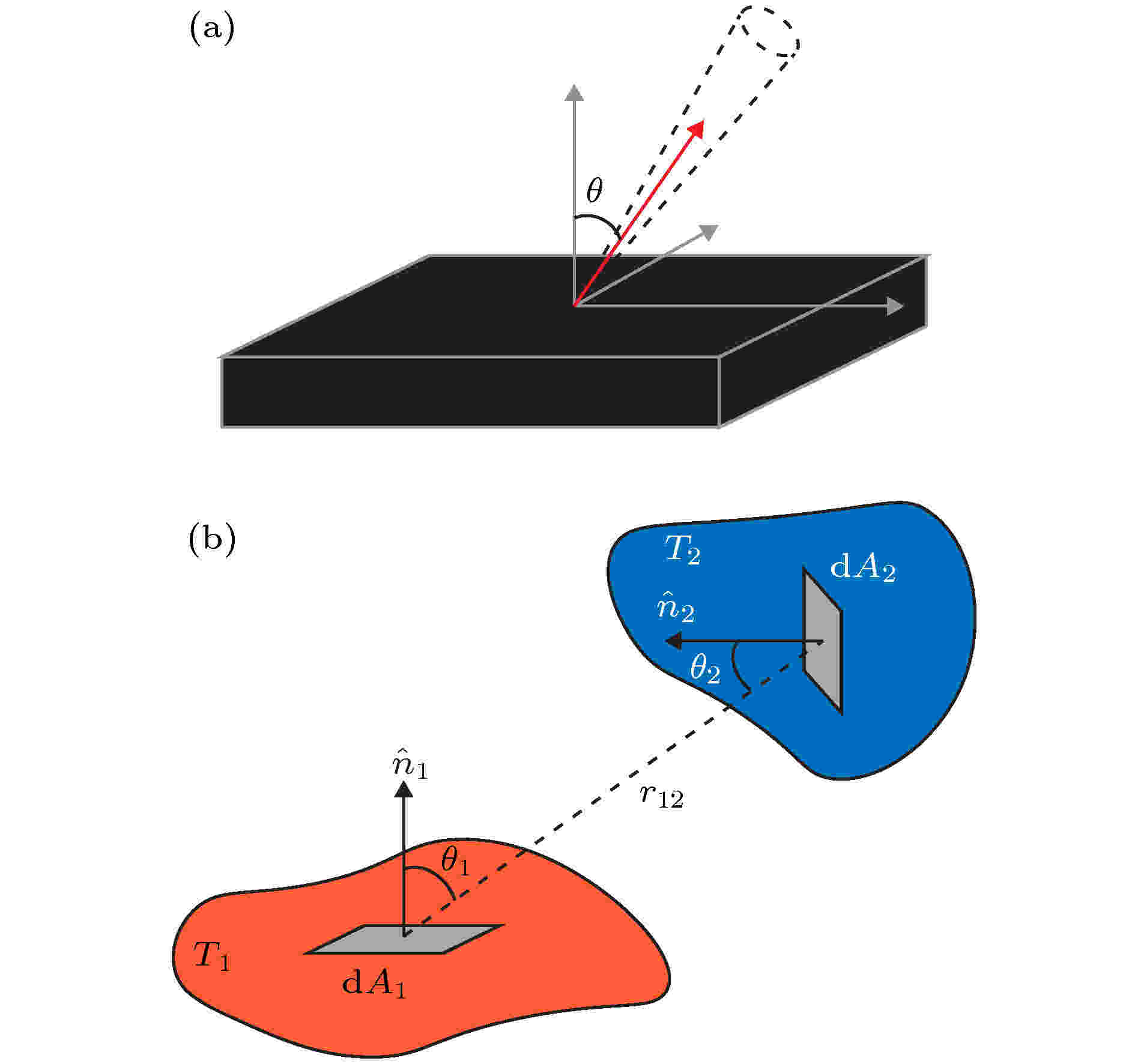## 封面文章

##### 领域
###### 特邀综述## 封面文章

2020, 69 (3): 036501. doi: 10.7498/aps.69.20191906

###### 综述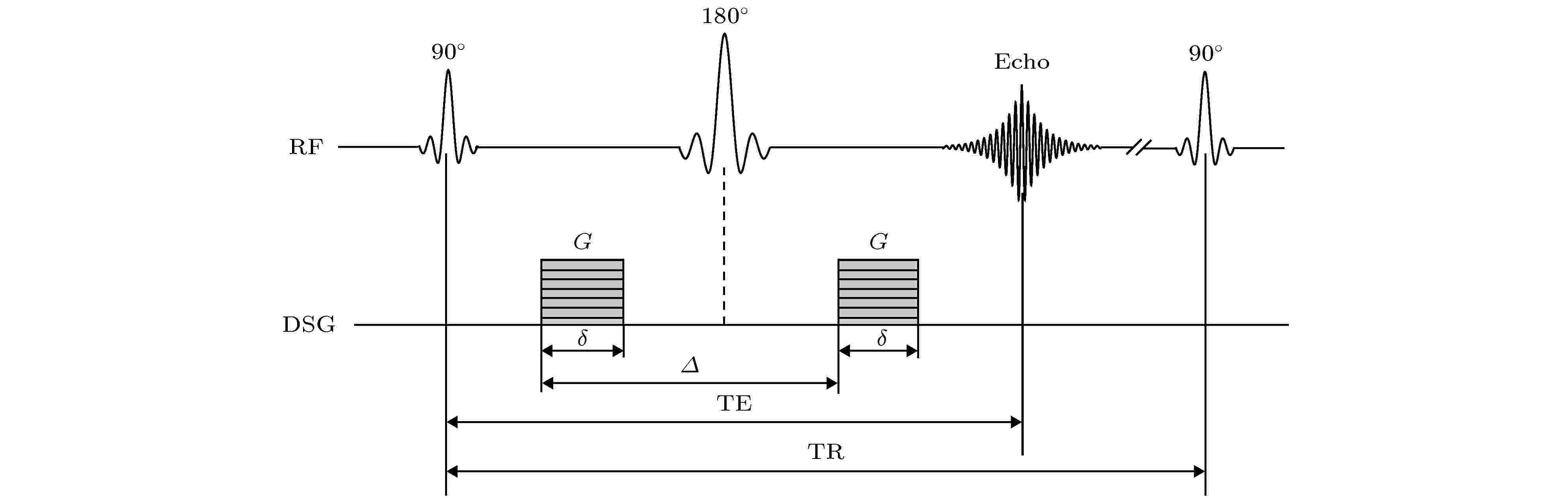2020, 69 (3): 038702. doi: 10.7498/aps.69.20191346

###### 总论2020, 69 (3): 030301. doi: 10.7498/aps.69.20191173

${\mathcal{P}}{\mathcal{T}}$-对称量子系统), 其哈密顿不是自伴的, 这类系统称为非自伴量子系统. 为了深入研究${\mathcal{P}}{\mathcal{T}}$-对称量子系统, 并考虑到算子${\mathcal{P}}{\mathcal{T}}$的共轭线性性, 首先讨论了共轭线性算子的一些性质, 包括它们的矩阵表示和谱结构等; 其次, 分别研究了具有共轭线性对称性和完整共轭线性对称性的线性算子, 通过它们的矩阵表示, 给出了共轭线性对称性和完整共轭线性对称性的等价刻画; 作为应用, 得到了关于${\mathcal{P}}{\mathcal{T}}$-对称及完整${\mathcal{P}}{\mathcal{T}}$-对称算子的一些有趣性质, 并通过一些具体例子, 说明了完整${\mathcal{P}}{\mathcal{T}}$-对称性对张量积运算不具有封闭性, 同时说明了完整${\mathcal{P}}{\mathcal{T}}$-对称性既不是哈密顿算子在某个正定内积下自伴的充分条件, 也不是必要条件.">传统量子系统的哈密顿是自伴算子, 哈密顿的自伴性不仅保证系统遵循酉演化和保持概率守恒, 而且也保证了它自身具有实的能量本征值, 这类系统称为自伴量子系统. 然而, 确实存在一些物理系统(如${\mathcal{P}}{\mathcal{T}}$-对称量子系统), 其哈密顿不是自伴的, 这类系统称为非自伴量子系统. 为了深入研究${\mathcal{P}}{\mathcal{T}}$-对称量子系统, 并考虑到算子${\mathcal{P}}{\mathcal{T}}$的共轭线性性, 首先讨论了共轭线性算子的一些性质, 包括它们的矩阵表示和谱结构等; 其次, 分别研究了具有共轭线性对称性和完整共轭线性对称性的线性算子, 通过它们的矩阵表示, 给出了共轭线性对称性和完整共轭线性对称性的等价刻画; 作为应用, 得到了关于${\mathcal{P}}{\mathcal{T}}$-对称及完整${\mathcal{P}}{\mathcal{T}}$-对称算子的一些有趣性质, 并通过一些具体例子, 说明了完整${\mathcal{P}}{\mathcal{T}}$-对称性对张量积运算不具有封闭性, 同时说明了完整${\mathcal{P}}{\mathcal{T}}$-对称性既不是哈密顿算子在某个正定内积下自伴的充分条件, 也不是必要条件.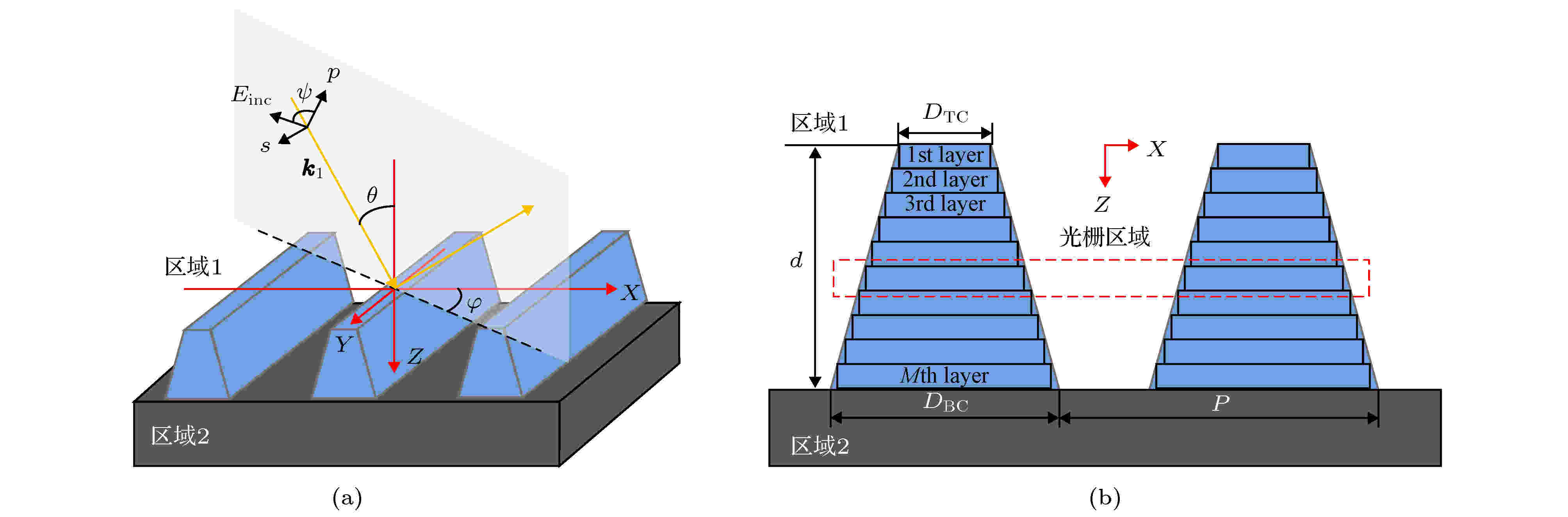## 编辑推荐

2020, 69 (3): 030601. doi: 10.7498/aps.69.20191525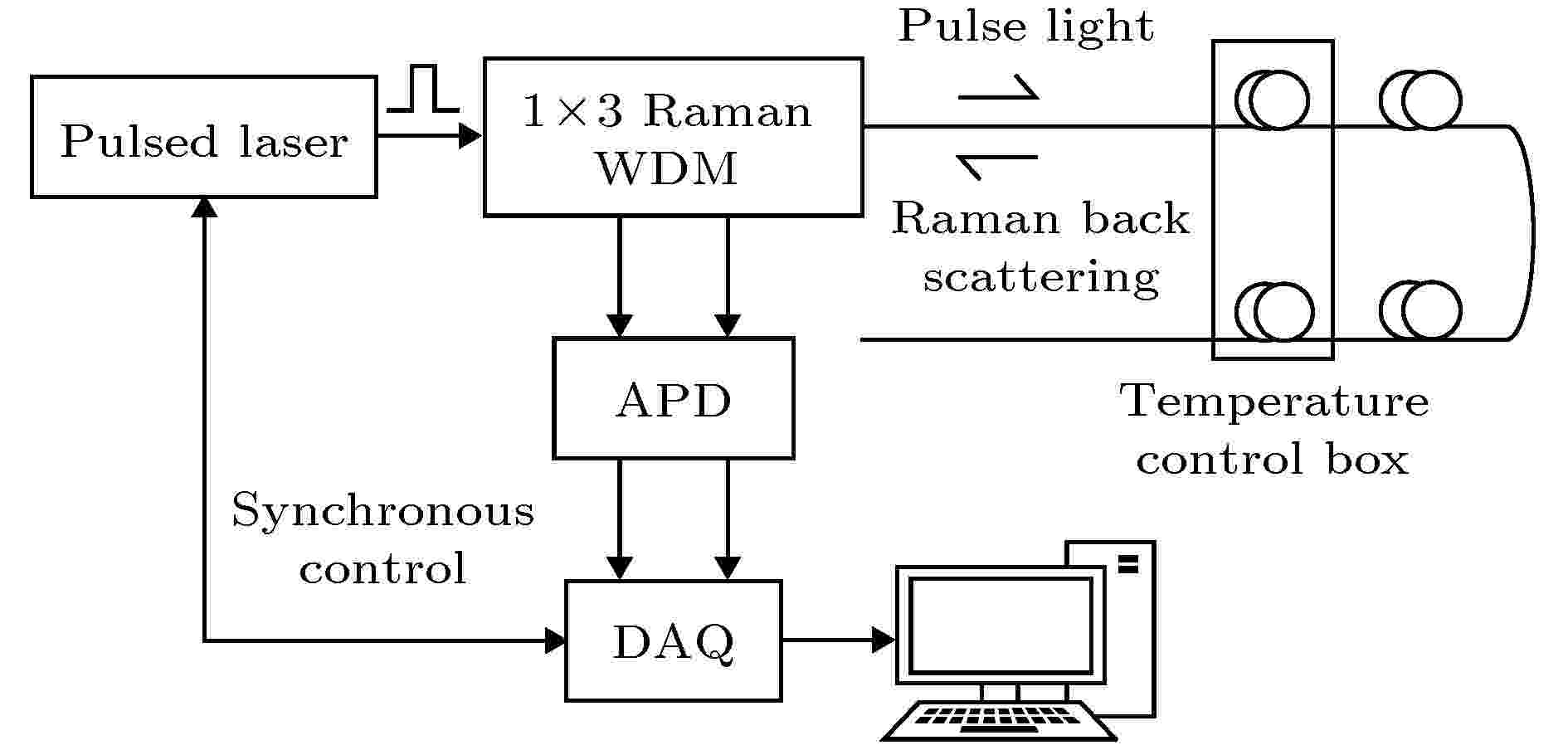2020, 69 (3): 030701. doi: 10.7498/aps.69.20191456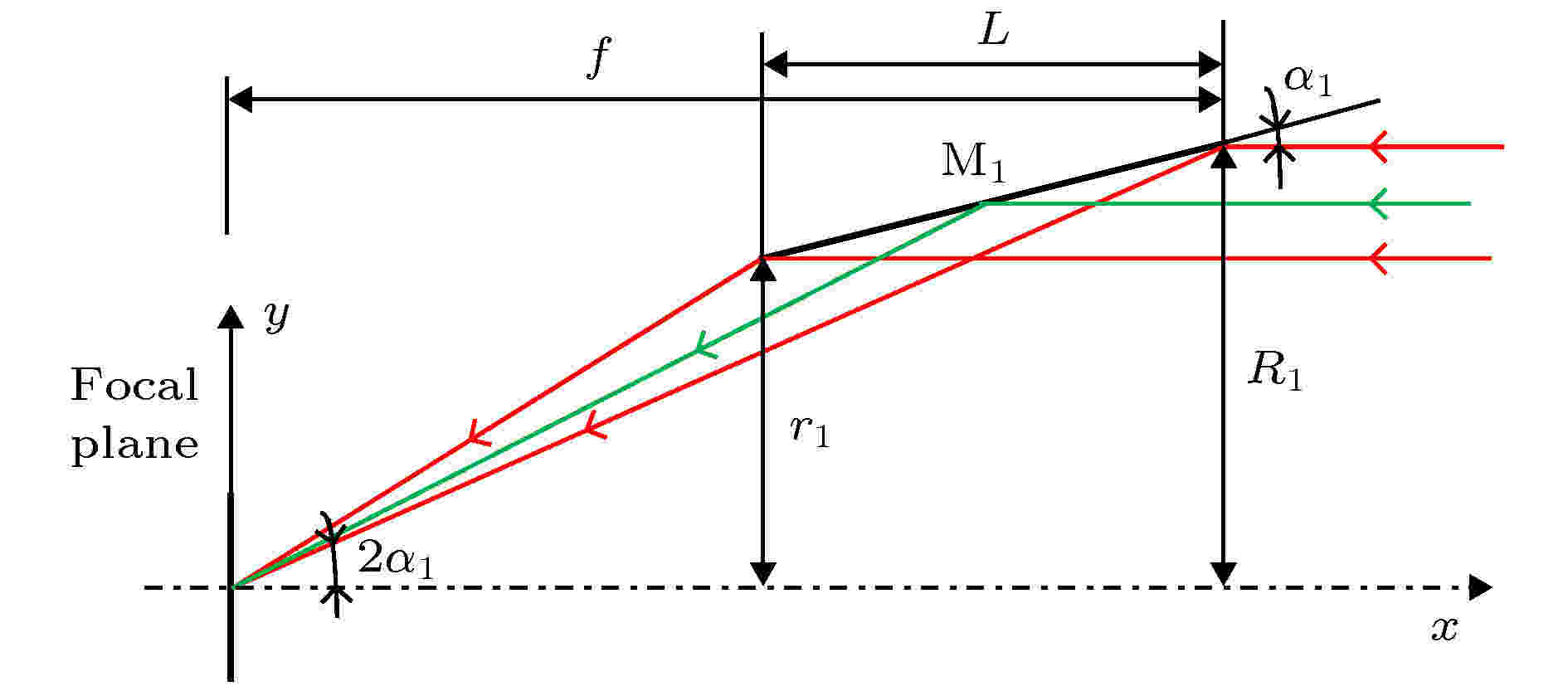2020, 69 (3): 030702. doi: 10.7498/aps.69.20191446

X射线掠入射光学系统是我国首颗脉冲星导航试验卫星主载荷聚焦型脉冲星探测器的核心部件, 在增大探测面积、提高探测器灵敏度方面发挥着重要作用, 实现了国内首次在轨验证. 针对脉冲星导航探测X射线光子到达时间的特点, 开展了基于单次抛物面镜反射的掠入射聚焦光学系统设计, 通过理论计算与推导, 获得了可制造的光学系统反射镜设计参数, 光学系统理论有效面积为15.6 cm2@1 keV, 对设计的光学系统进行了聚焦性能仿真, 全视场范围内均满足探测器聚焦要求, 开展电铸镍复制工艺研究, 完成了芯轴的超精密控形加工, 在此基础上制造了4层金属反射镜, 利用北京同步辐射4B7B光束线测试了各层反射镜的反射率, 基于实测反射率的光学系统有效面积为13.2 cm2@1 keV. 最后基于在轨观测数据, 评价得到光学系统的有效面积为4.22 cm2@1 keV, 分析了地面标定有效面积与在轨评价有效面积存在差别的原因, 验证了设计、仿真与制造方法的正确性, 为大面积掠入射光学系统的研制奠定了基础.
###### 核物理学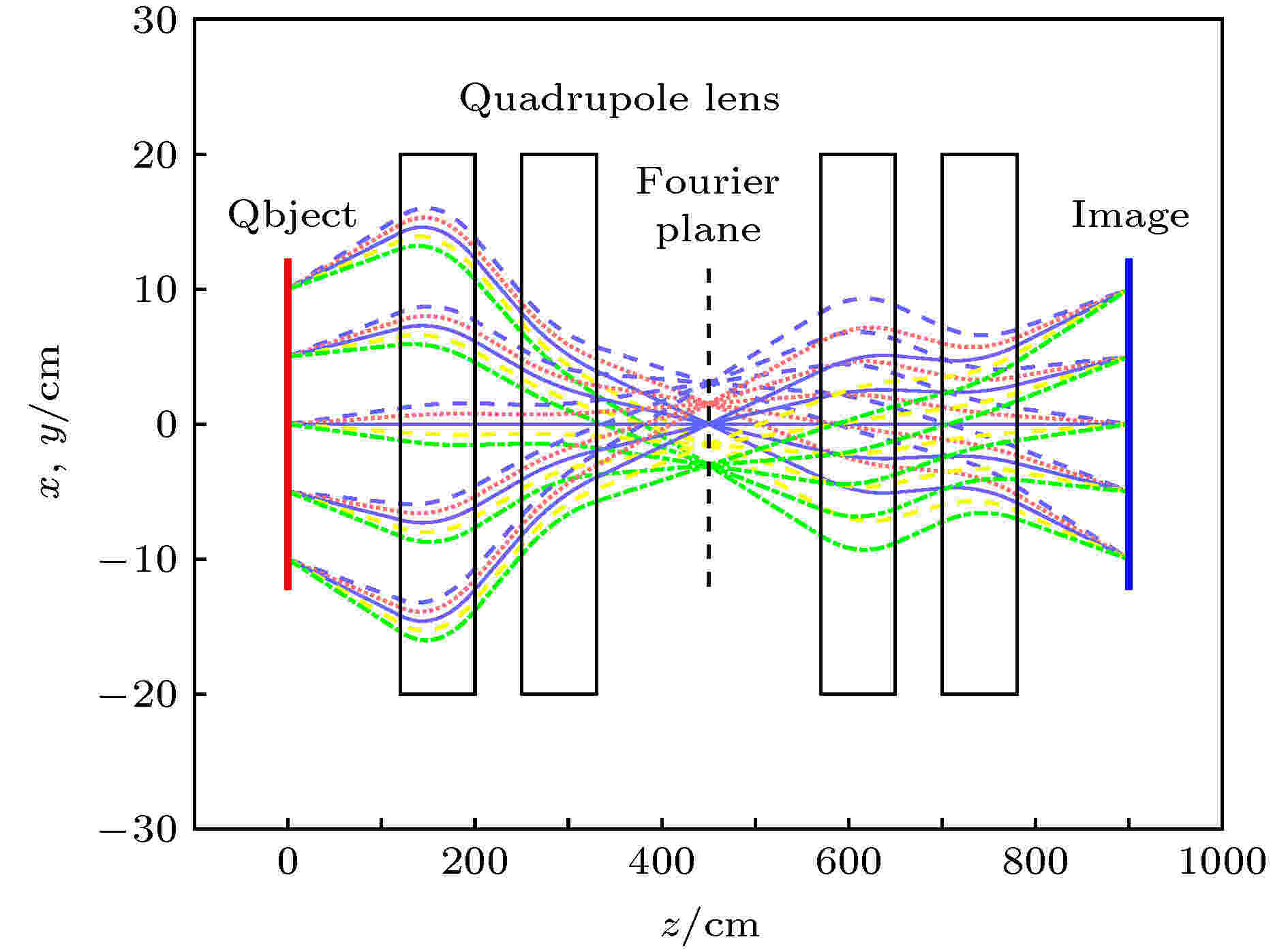2020, 69 (3): 032901. doi: 10.7498/aps.69.20191691

###### 原子和分子物理学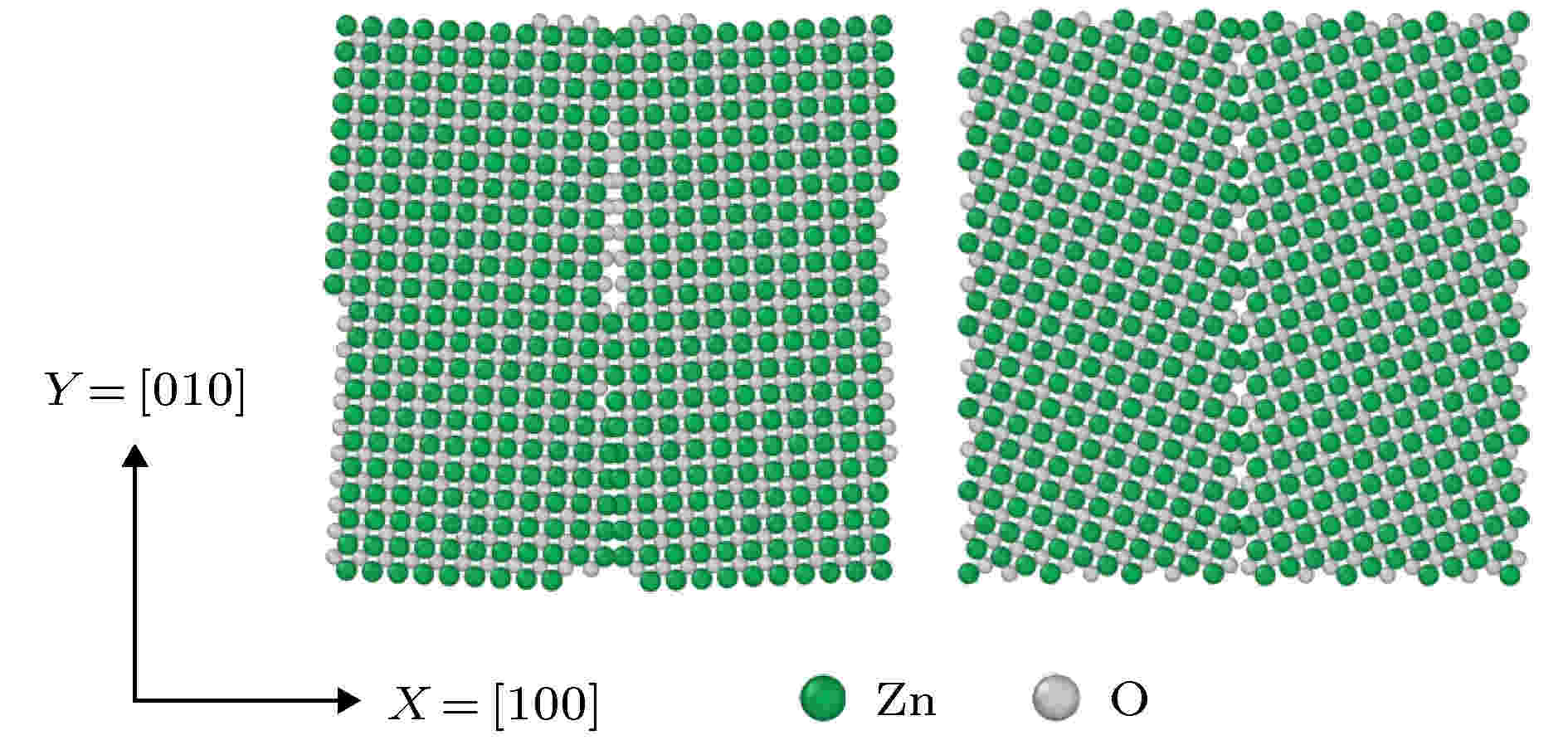2020, 69 (3): 033101. doi: 10.7498/aps.69.20190627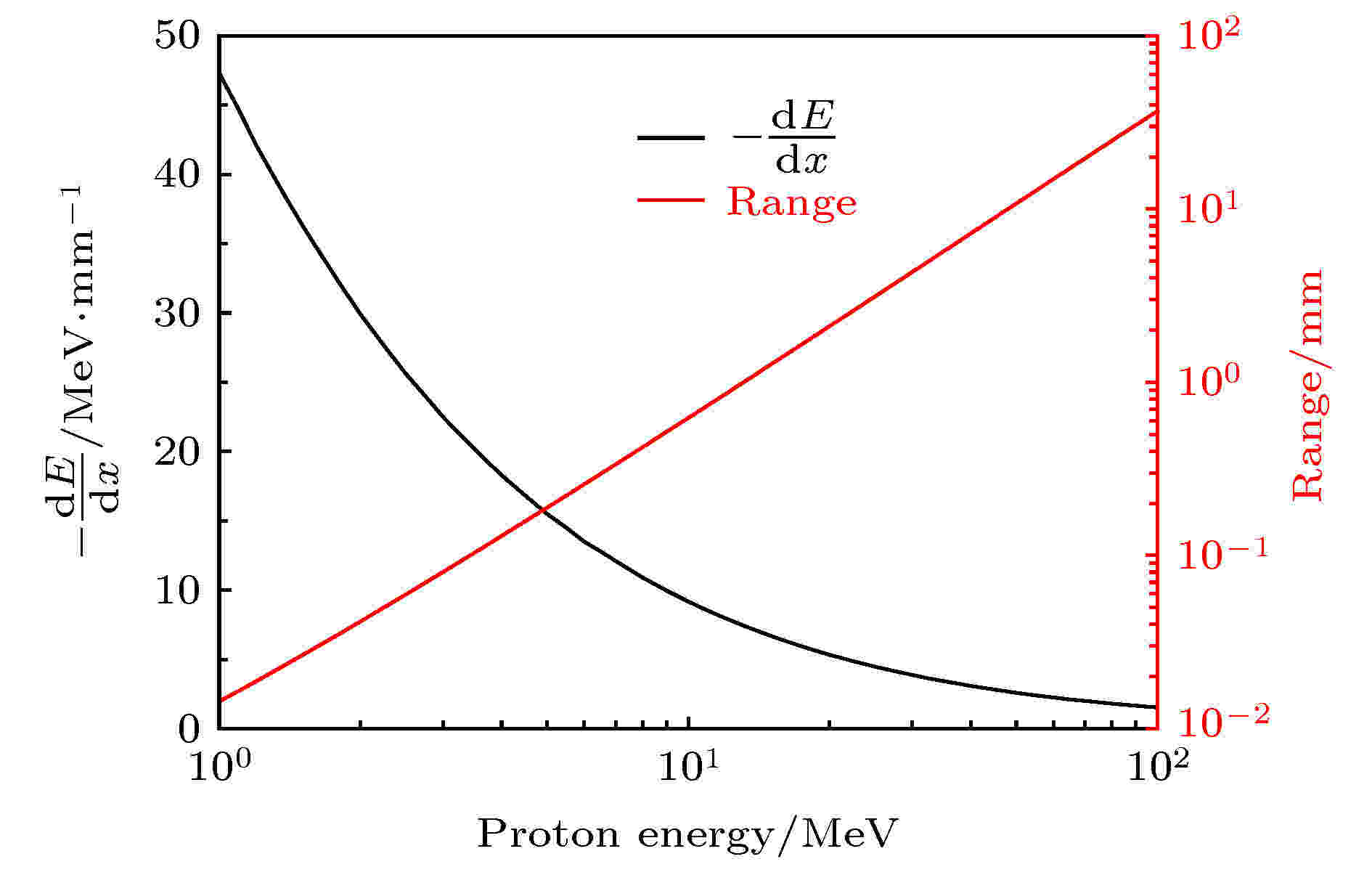2020, 69 (3): 033401. doi: 10.7498/aps.69.20191514

###### 电磁学、光学、声学、传热学、经典力学和流体动力学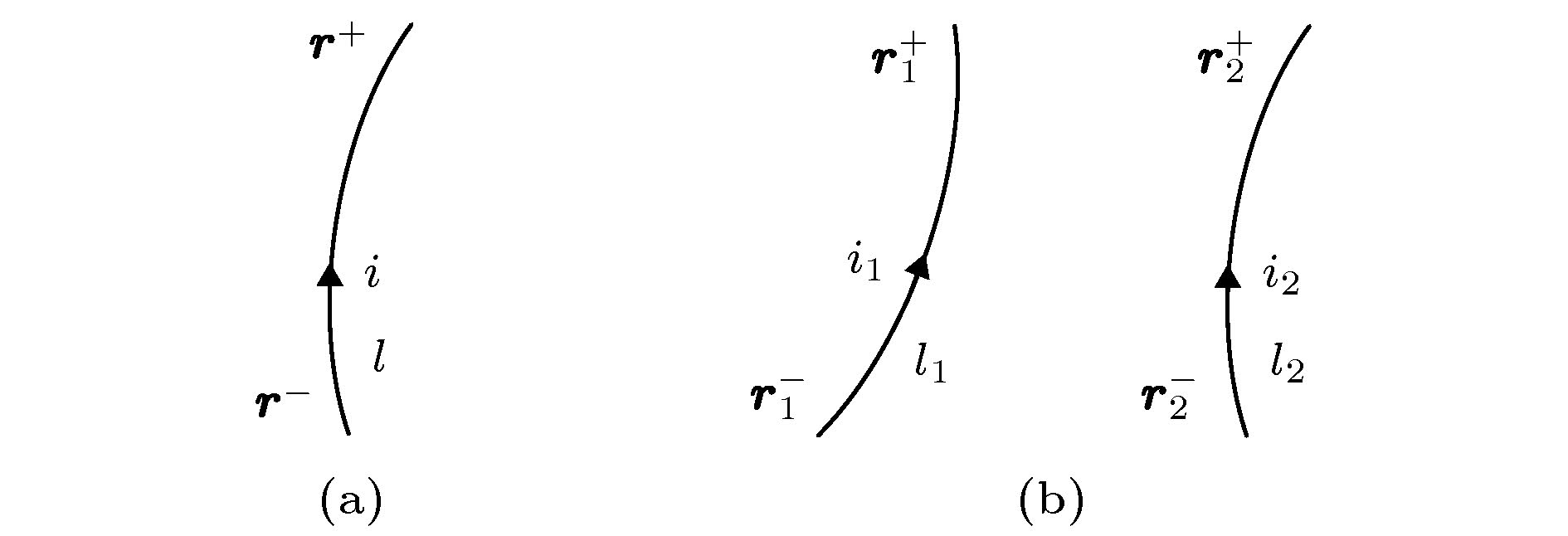2020, 69 (3): 034101. doi: 10.7498/aps.69.20191212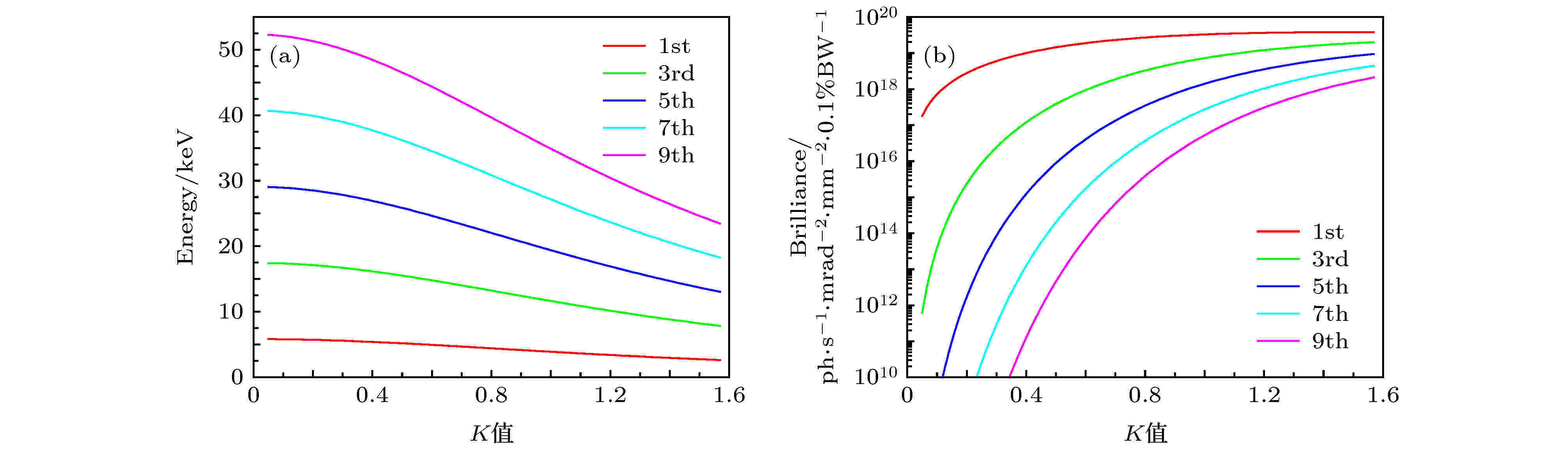2020, 69 (3): 034102. doi: 10.7498/aps.69.20191586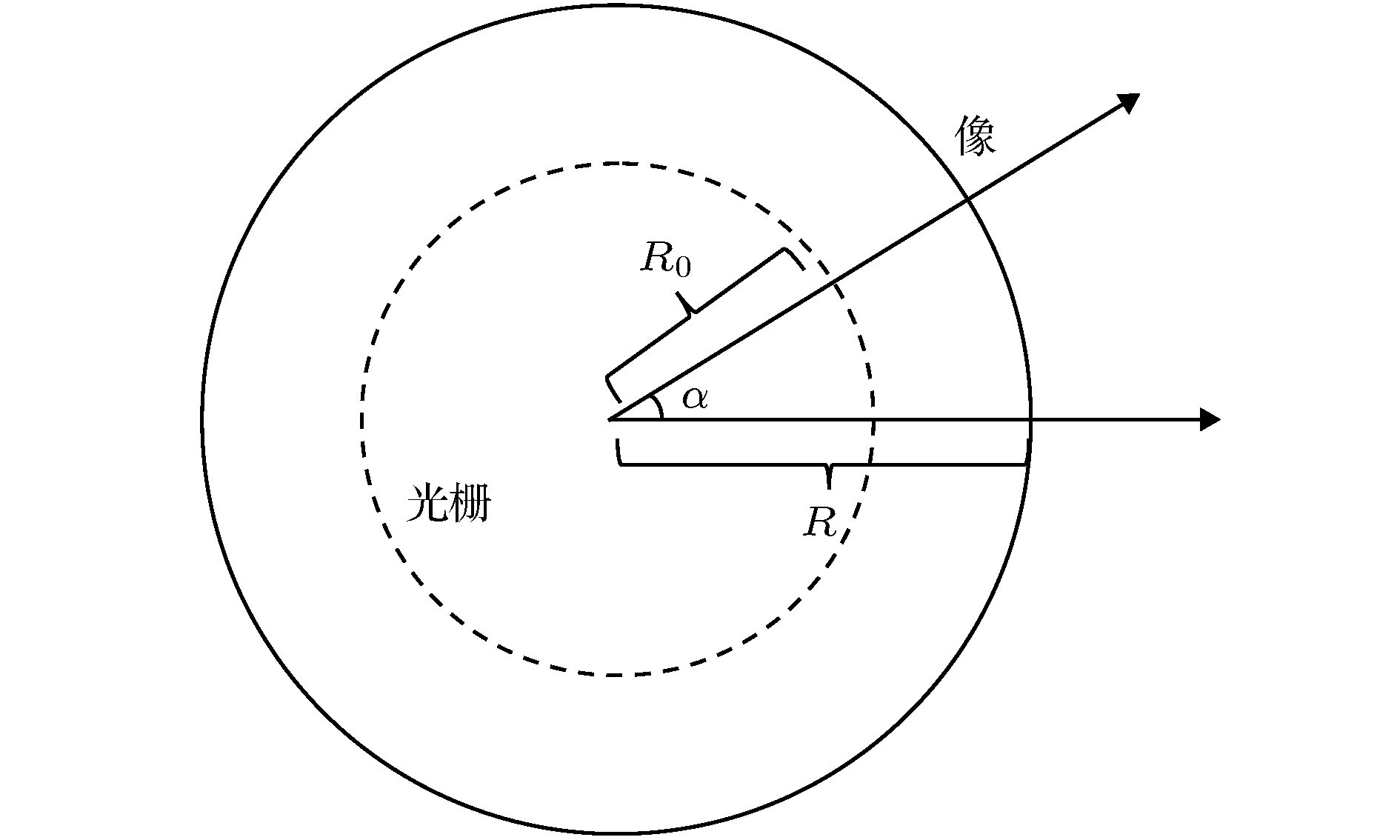2020, 69 (3): 034201. doi: 10.7498/aps.69.20191340

Talbot效应是一种近场自成像效应, 通常只有周期光栅可以产生Talbot效应, 而环形光栅无法产生. 本文通过引入保角变换, 发现可以在环形光栅外部设计适当的折射率渐变层介质, 使得其中也能够产生严格的Talbot效应, 并计算了对应的自成像半径表达式. 本文利用FDTD软件分别将一个环形光栅放置在真空中以及人工设计的折射率渐变层中进行了模拟, 并对二者的结果进行了比较分析, 发现这种折射率渐变层介质确实对点光源入射的环形光栅的自成像情况有着很好的改善. 希望这一工作能够推广Talbot效应的应用范围.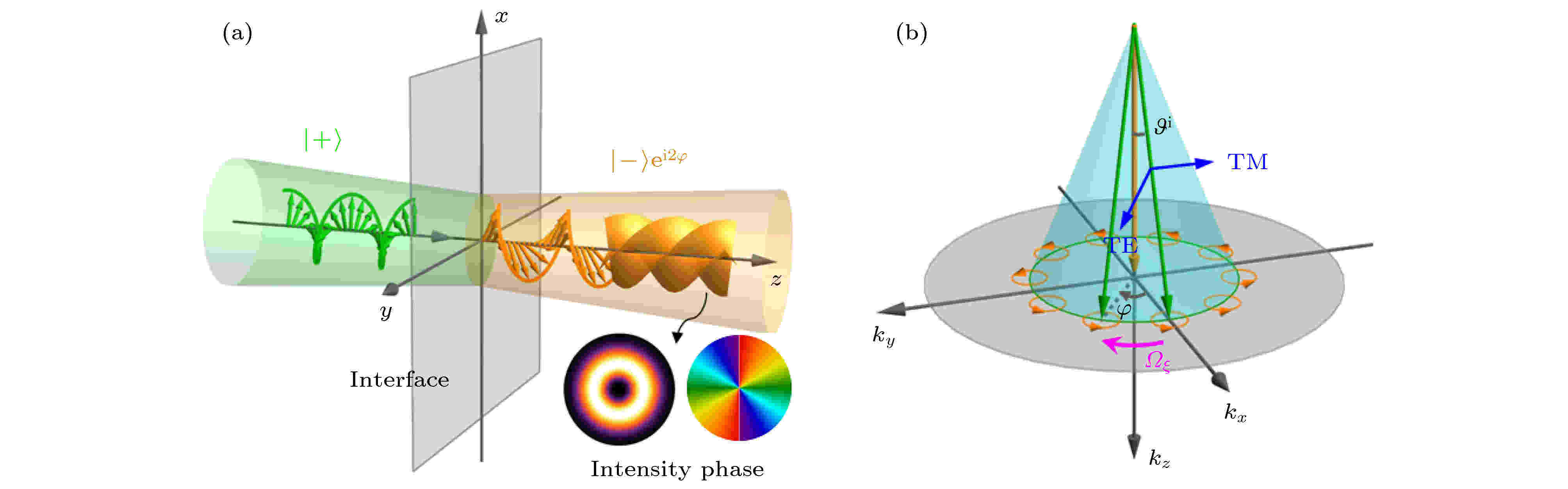2020, 69 (3): 034202. doi: 10.7498/aps.69.201912182020, 69 (3): 034203. doi: 10.7498/aps.69.20191143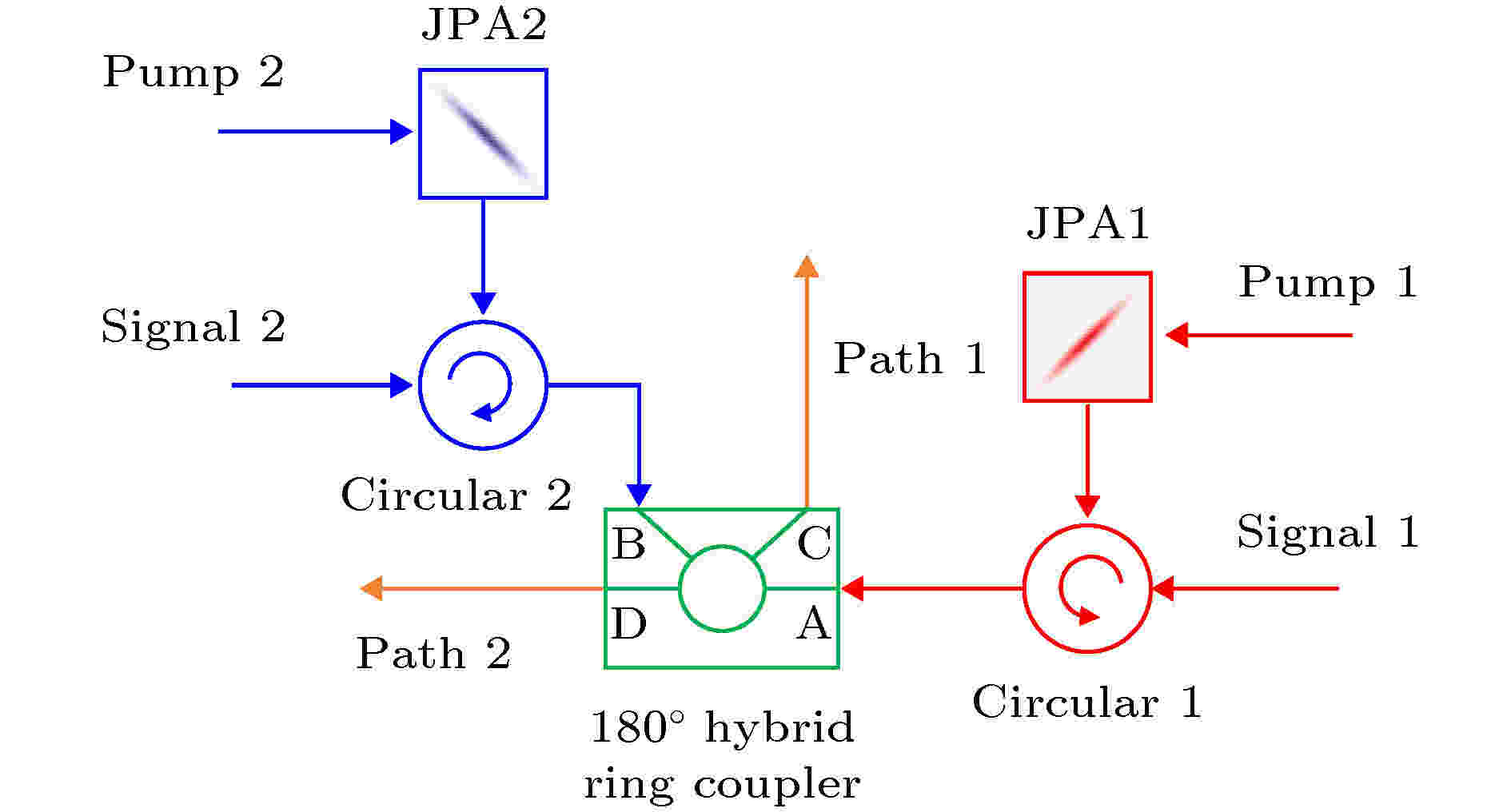2020, 69 (3): 034204. doi: 10.7498/aps.69.20191348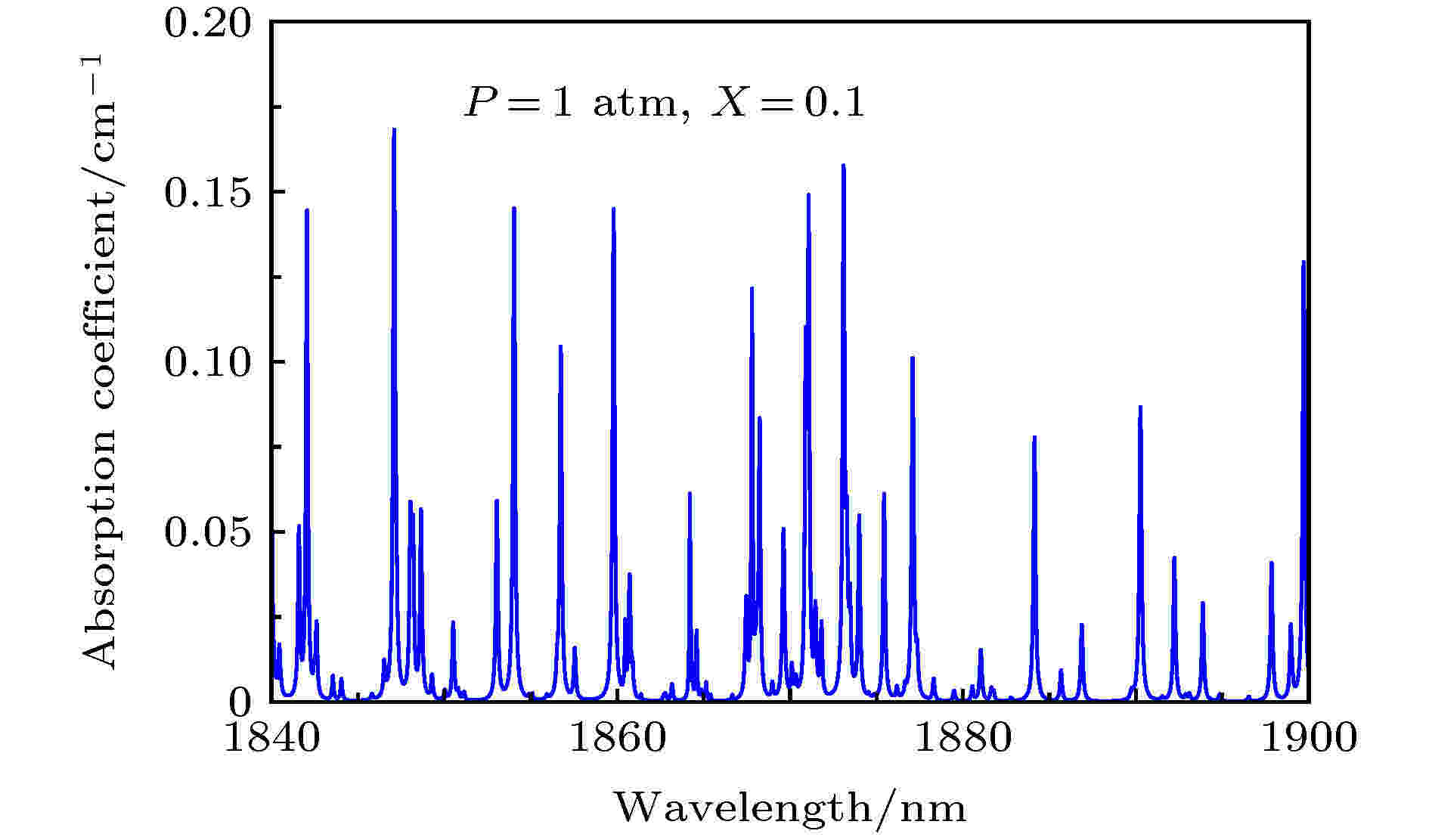## 编辑推荐

2020, 69 (3): 034205. doi: 10.7498/aps.69.20191515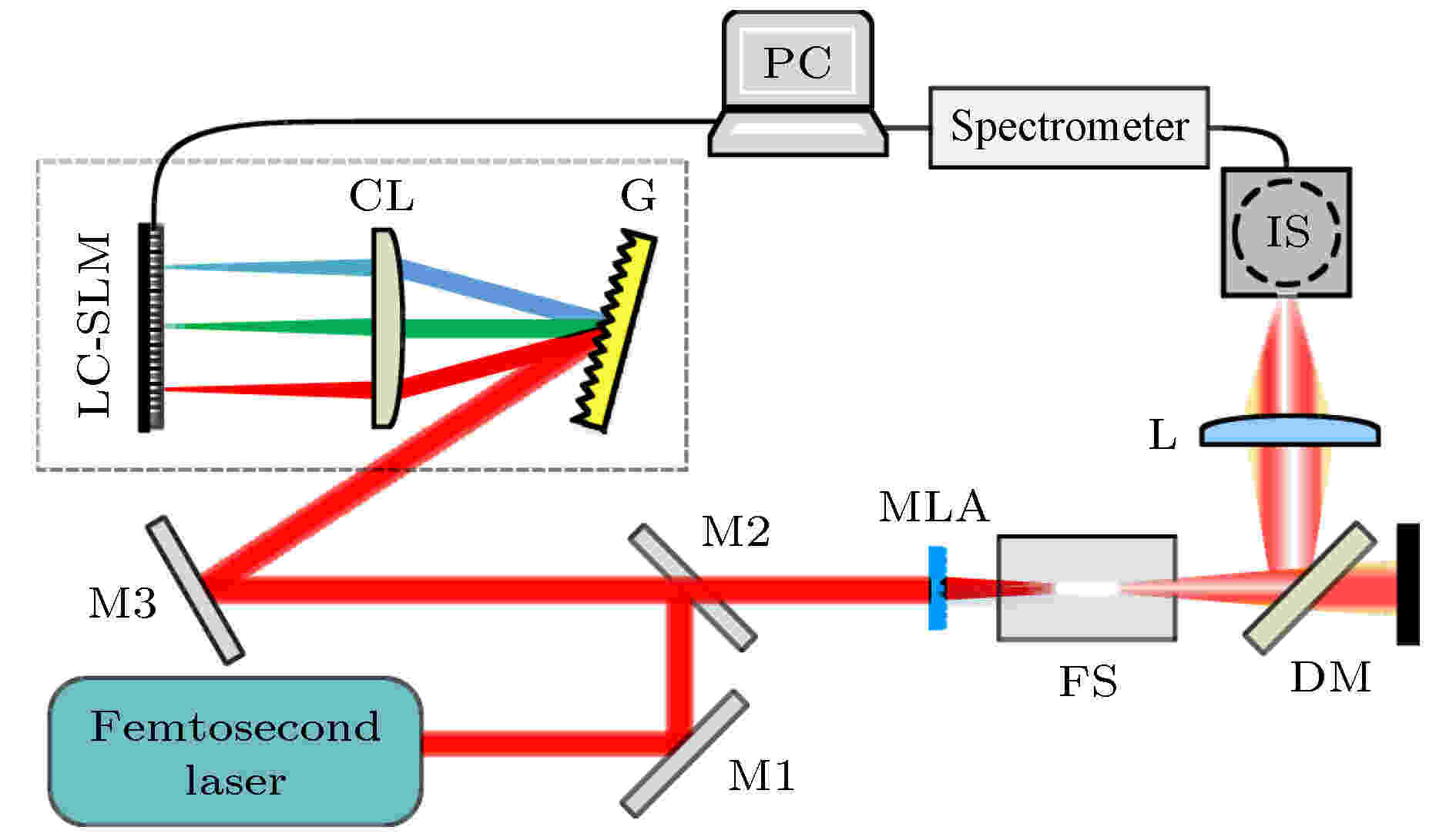2020, 69 (3): 034206. doi: 10.7498/aps.69.20191438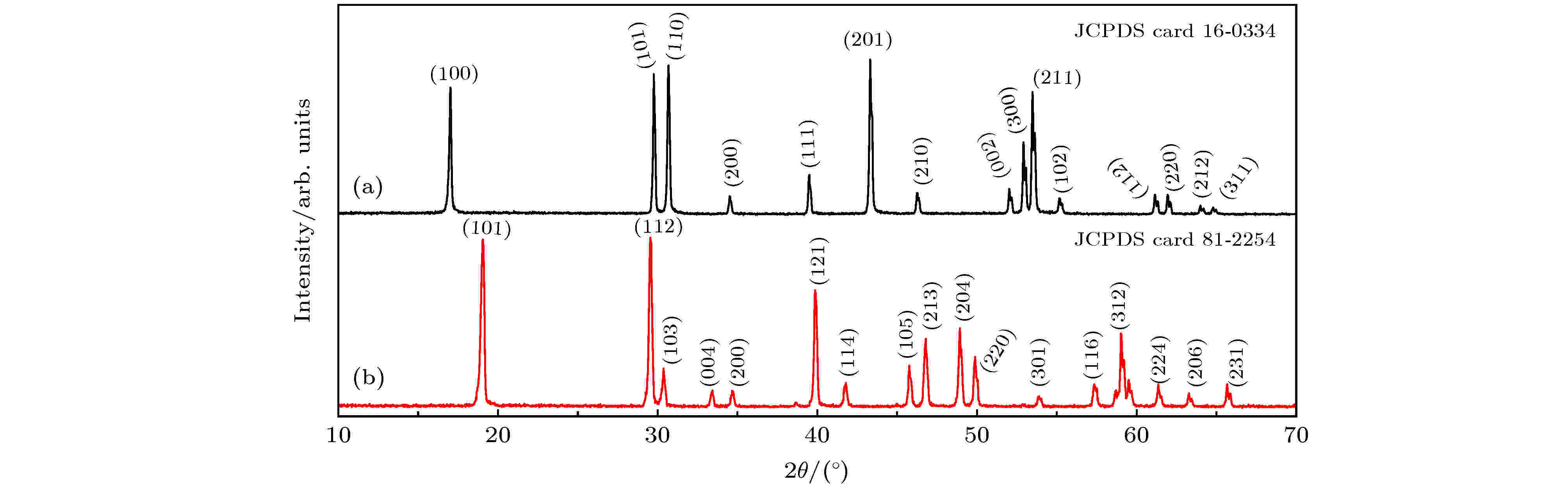2020, 69 (3): 034207. doi: 10.7498/aps.69.20191333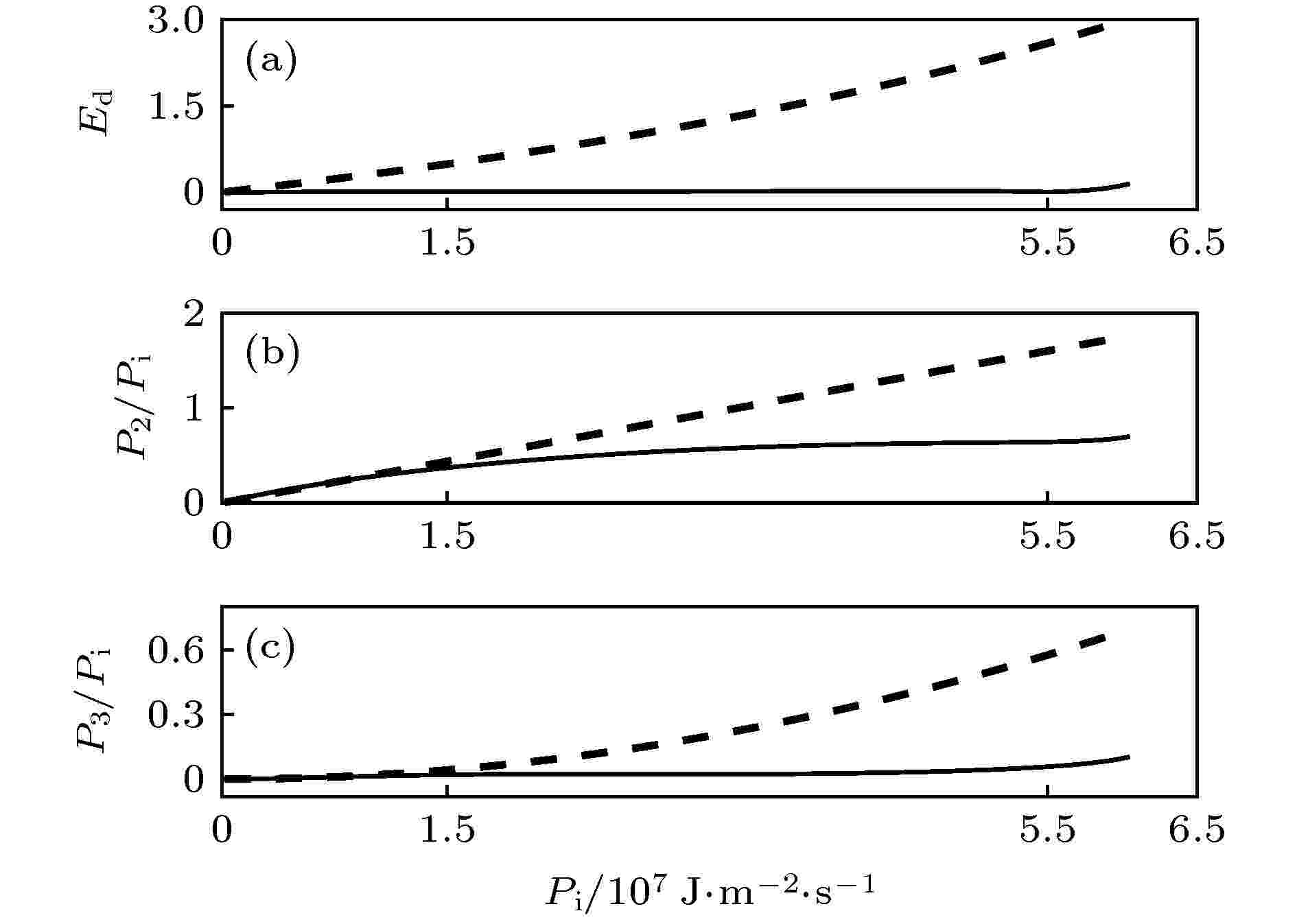2020, 69 (3): 034301. doi: 10.7498/aps.69.20191440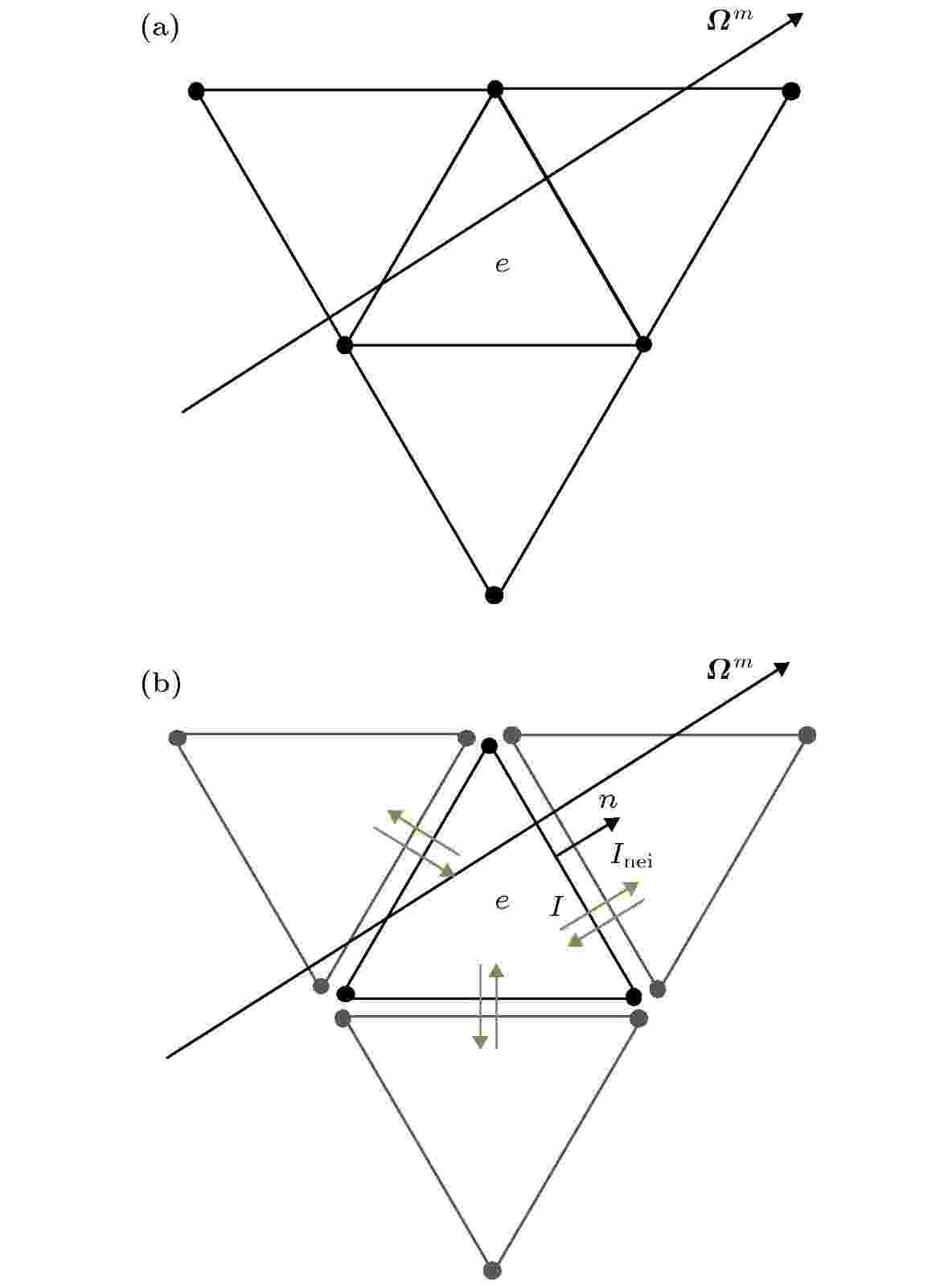2020, 69 (3): 034401. doi: 10.7498/aps.69.20191185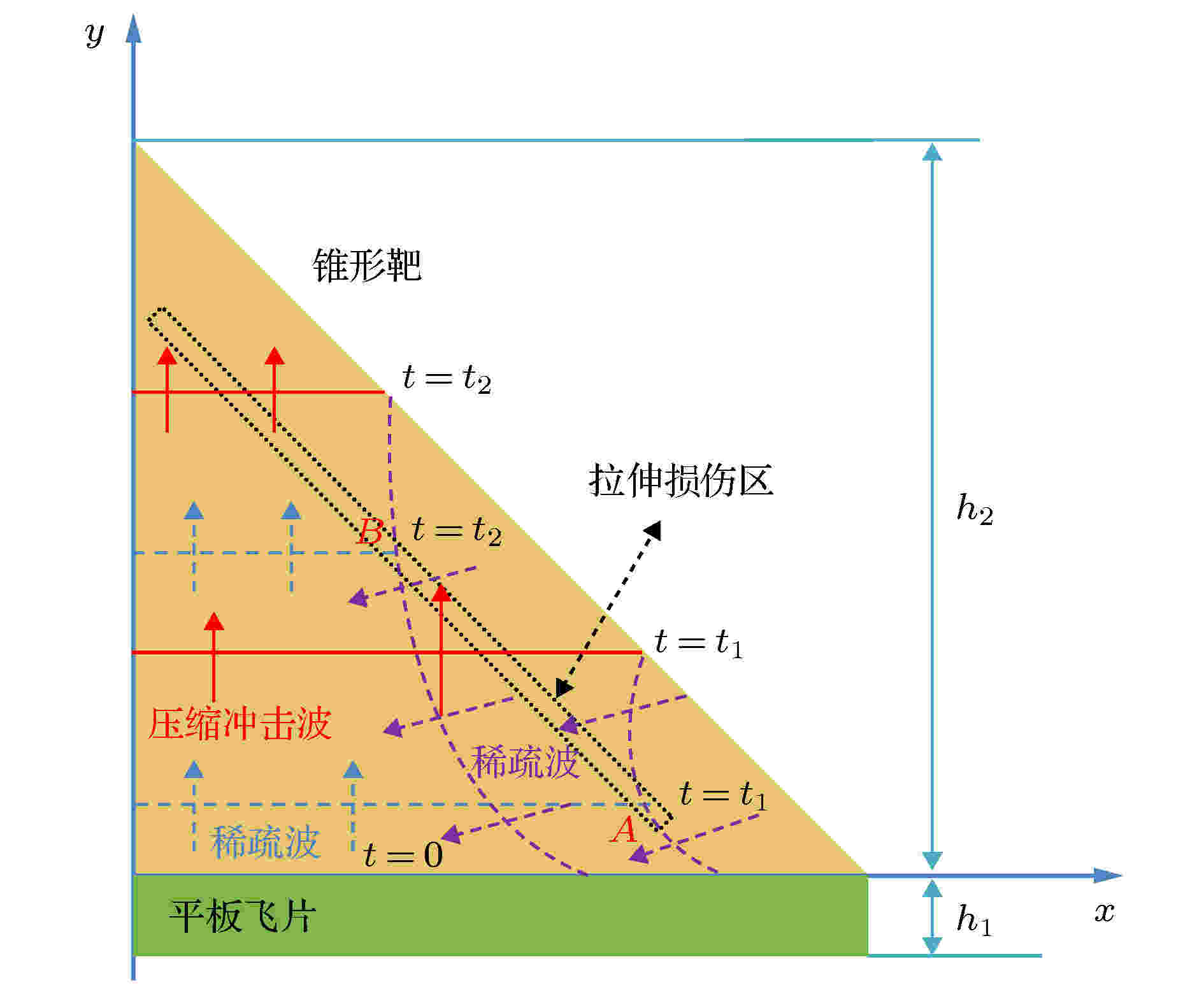2020, 69 (3): 034601. doi: 10.7498/aps.69.20191104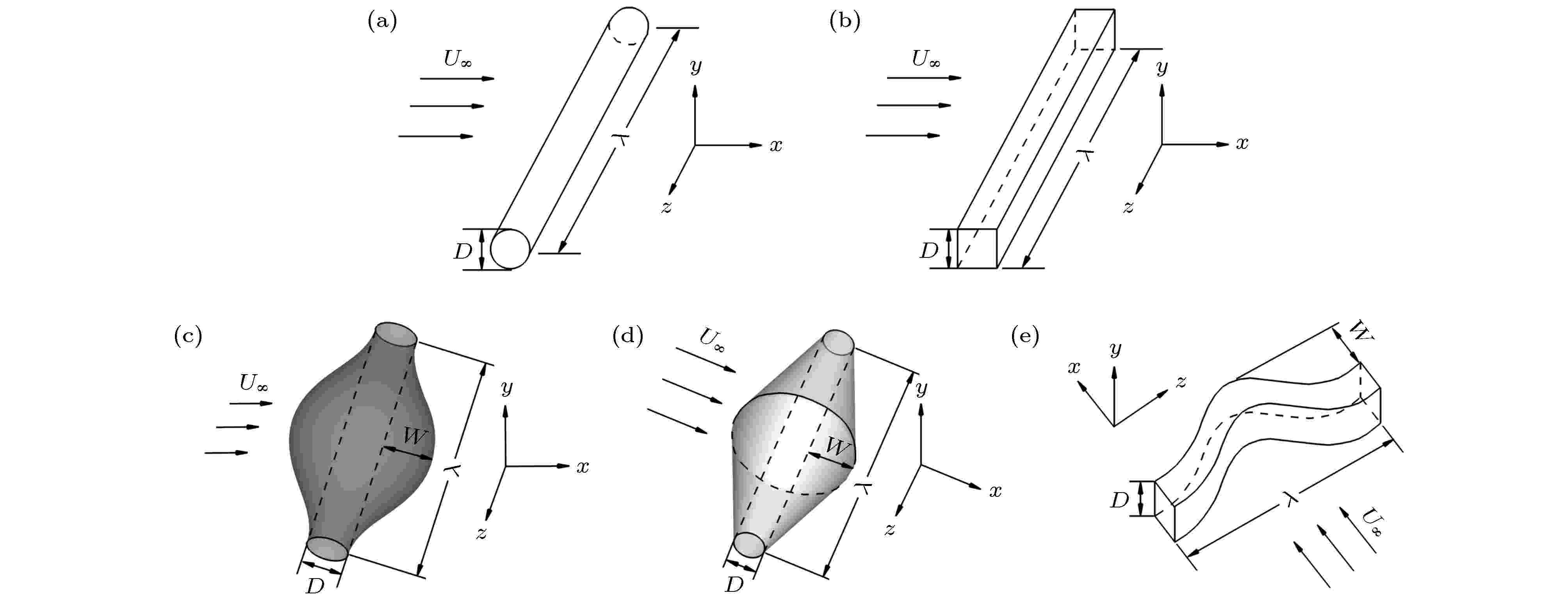## 编辑推荐

2020, 69 (3): 034701. doi: 10.7498/aps.69.20191011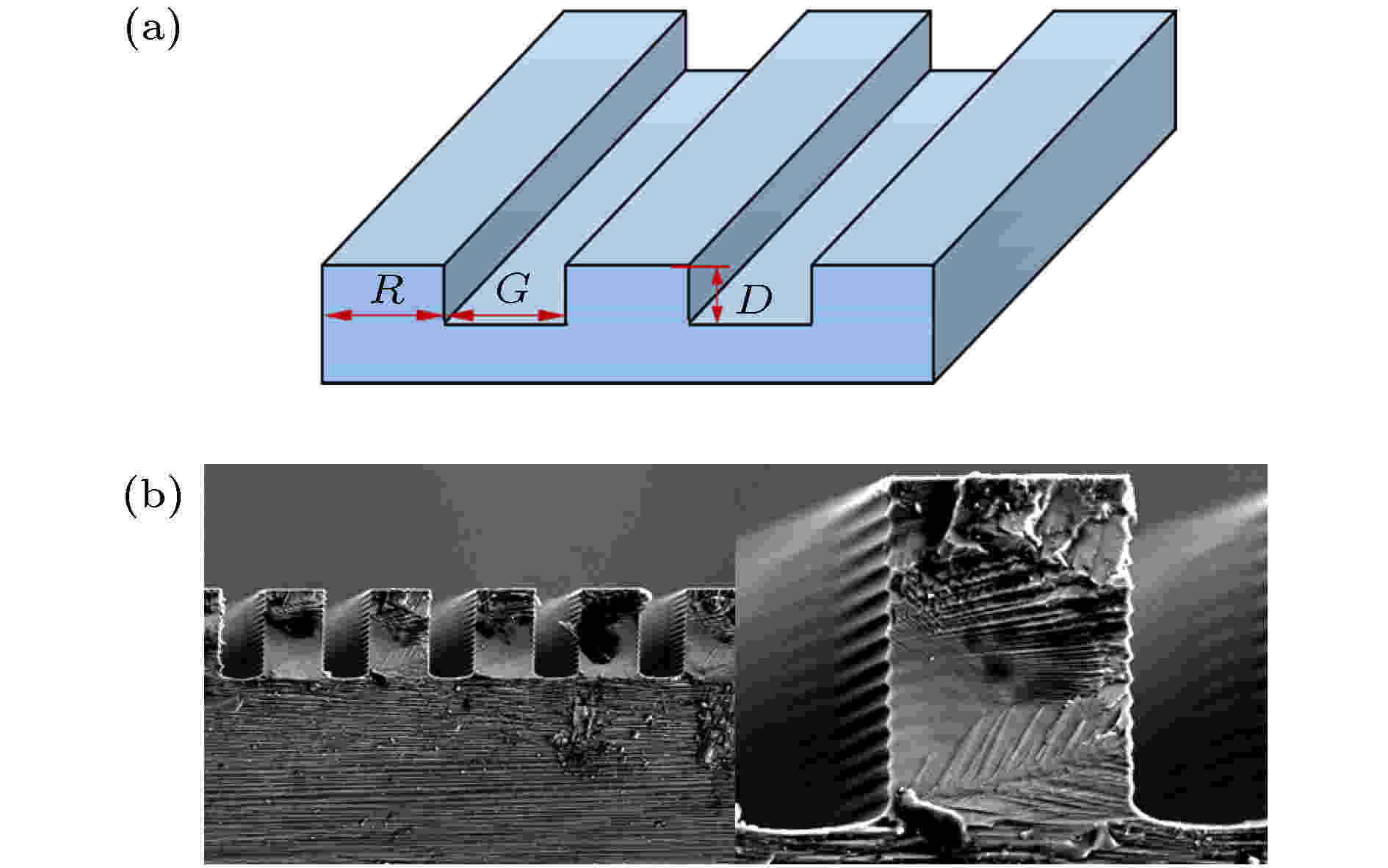2020, 69 (3): 034702. doi: 10.7498/aps.69.20191429

###### 气体、等离子体和放电物理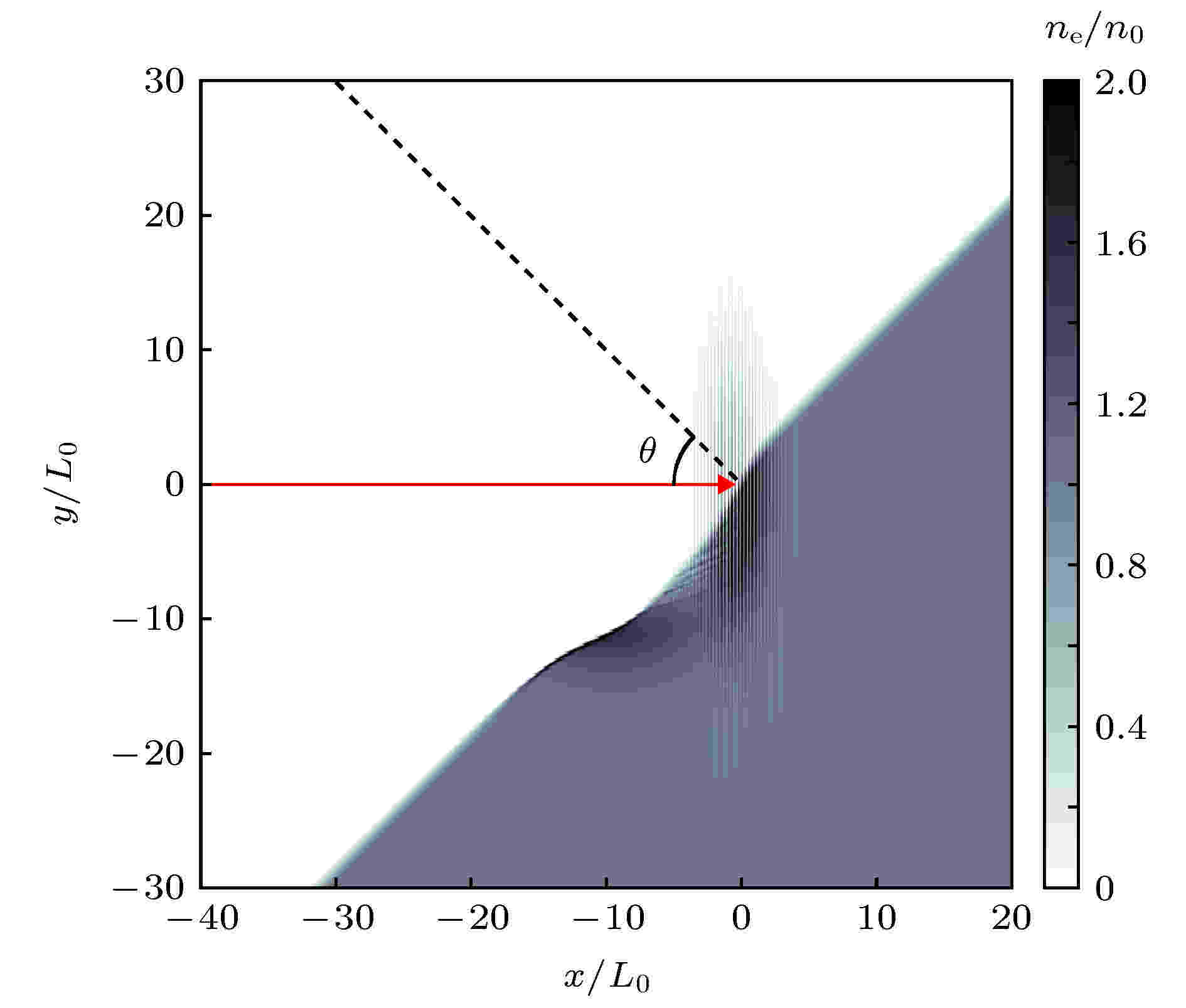2020, 69 (3): 035201. doi: 10.7498/aps.69.20191332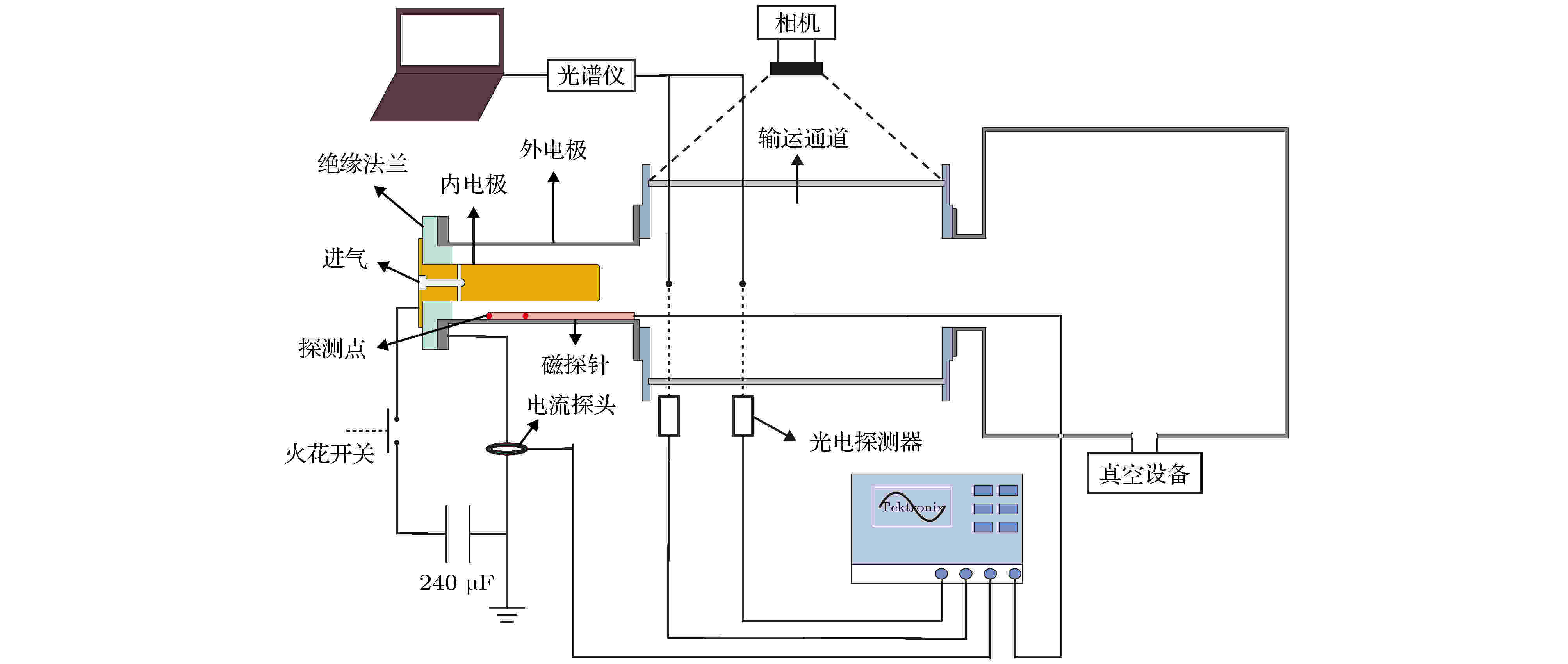2020, 69 (3): 035202. doi: 10.7498/aps.69.20191321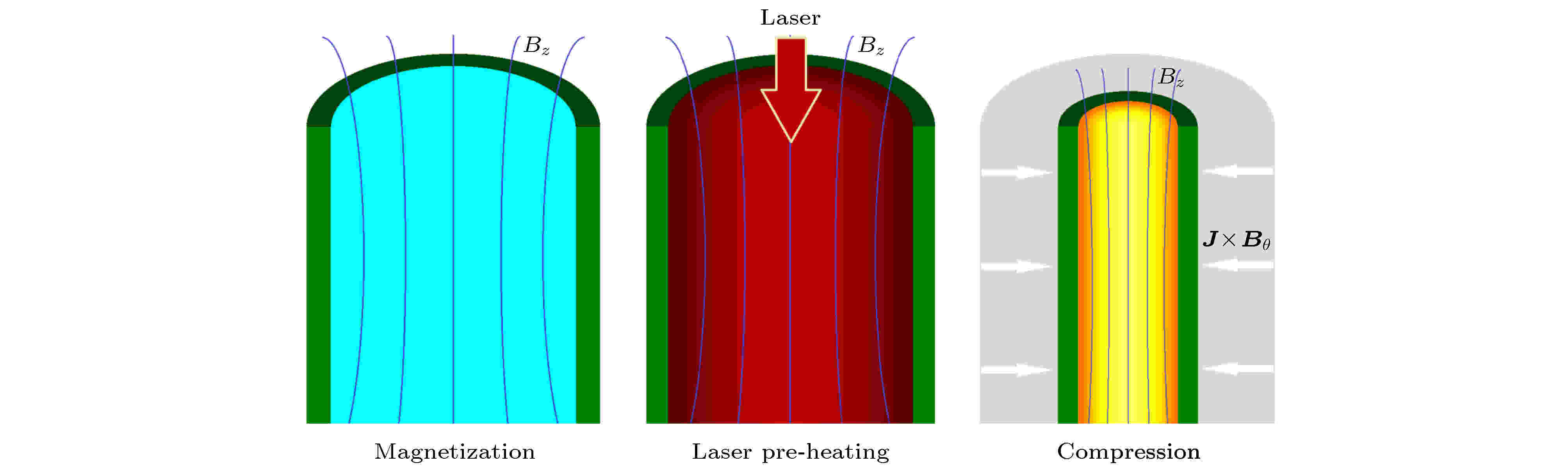2020, 69 (3): 035203. doi: 10.7498/aps.69.20191411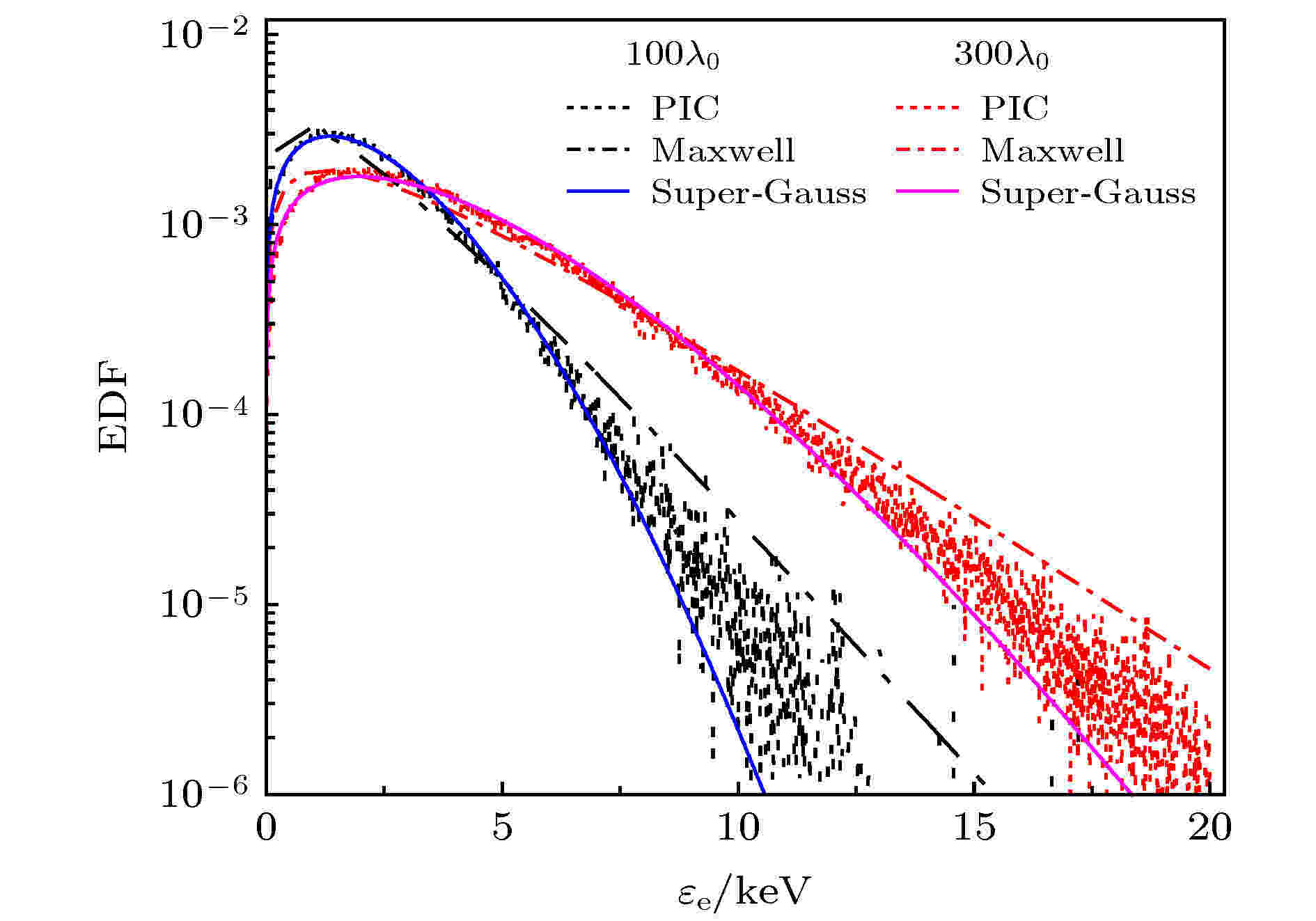2020, 69 (3): 035204. doi: 10.7498/aps.69.20191423

$\alpha=Z(v_{\rm{os}}/v_{\rm{te}})^2 > 1$, 电子分布函数表现为超高斯分布(m = 3.34), 克努森数$\lambda_{\rm{e}}/L_{\rm{e}}=0.011$大于局域S-H理论的临界值$2\times10^{-3}$. 这导致了局域S-H电子热流远大于实际热流. 这种实际热流受限现象将导致辐射流体模拟给出的冕区电子温度高于神光实验测量值. 而在等离子体的高密度区域, 电子分布函数仍表现为超高斯分布(m = 2.93), 克努森数$\lambda_{\rm{e}}/L_{\rm{e}}=7.58\times10^{-4}$小于局域S-H理论的临界值, 限流的局域S-H电子热流具有一定的适用性. 但电子热流严重依赖于限流因子$f_{\rm{e}}$, 辐射流体模拟需要根据不同位置的光强和电子温度调整$f_{\rm e}$的大小.">辐射流体采用限流的局域Spitzer-Härm (S-H)电子热流近似, 在预估等离子体状态时可能与实验观察存在偏差. 利用一维(1D3V)含碰撞的粒子模拟程序, 研究了激光聚变黑腔中金等离子体的电子分布函数和电子热流. 分析表明, 在等离子体的冕区, $\alpha=Z(v_{\rm{os}}/v_{\rm{te}})^2 > 1$, 电子分布函数表现为超高斯分布(m = 3.34), 克努森数$\lambda_{\rm{e}}/L_{\rm{e}}=0.011$大于局域S-H理论的临界值$2\times10^{-3}$. 这导致了局域S-H电子热流远大于实际热流. 这种实际热流受限现象将导致辐射流体模拟给出的冕区电子温度高于神光实验测量值. 而在等离子体的高密度区域, 电子分布函数仍表现为超高斯分布(m = 2.93), 克努森数$\lambda_{\rm{e}}/L_{\rm{e}}=7.58\times10^{-4}$小于局域S-H理论的临界值, 限流的局域S-H电子热流具有一定的适用性. 但电子热流严重依赖于限流因子$f_{\rm{e}}$, 辐射流体模拟需要根据不同位置的光强和电子温度调整$f_{\rm e}$的大小.
###### 凝聚物质：结构、力学和热学性质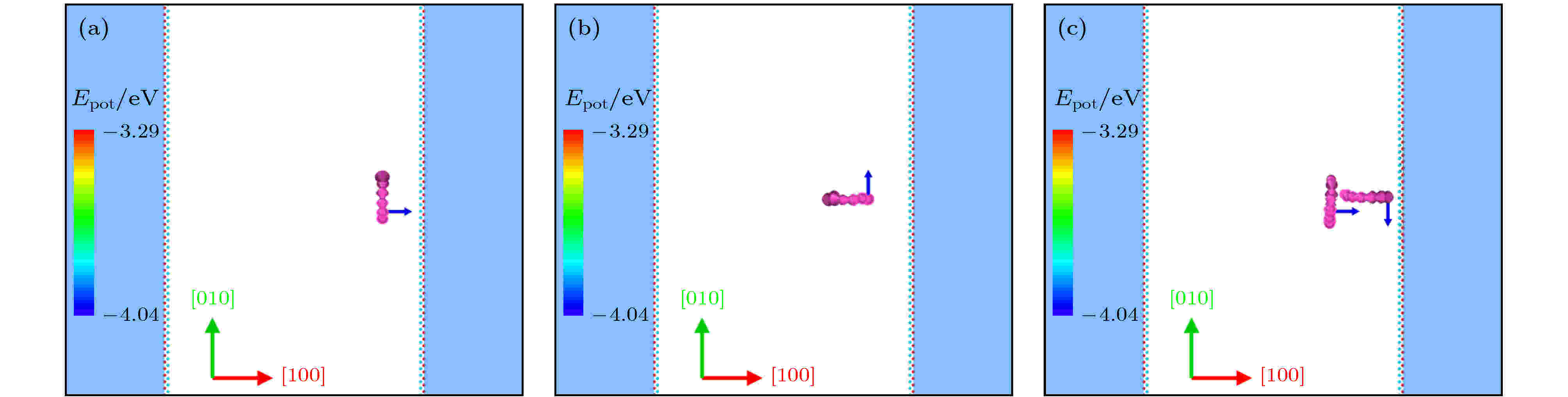2020, 69 (3): 036101. doi: 10.7498/aps.69.20191379

$\left\langle 111 \right\rangle$$\left\langle 100 \right\rangle$是两种主要的位错环, 其对辐照损伤的影响一直都是核材料领域研究的热点之一. 在之前的研究中, 人们对{111}面与单个1/2$\left\langle 111 \right\rangle$位错环的相互作用进行了深入研究, 发现表面对位错环性质确实有重要的影响. 采用分子动力学方法, 在原子尺度详细研究了另一个重要的表面铁{100}面对$\left\langle 100 \right\rangle$间隙型位错环动力学过程的影响. 模拟发现位错环伯格斯矢量与表面法线方向的关系、距表面的深度、位错环之间的相互作用以及温度等, 都对位错环与表面的相互作用产生重要影响, 其中, 表面作用下的伯格斯矢量的演化以及$\left\langle 100 \right\rangle$位错环在此过程中的一维运动首次被发现. 基于这些模拟结果, 就$\left\langle 100 \right\rangle$位错环对表面辐照损伤结构的影响进行详细地研究, 给出$\left\langle 100 \right\rangle$位错环对表面凹凸结构的贡献, 这些结果为理解辐照过程中材料表面的演化提供一种可能的解释.">在材料辐照损伤过程中, 间隙型位错环的形成及动力学行为严重影响材料在辐照条件下的服役行为. 在常用的以体心立方铁为基的合金材料中, 1/2$\left\langle 111 \right\rangle$$\left\langle 100 \right\rangle$是两种主要的位错环, 其对辐照损伤的影响一直都是核材料领域研究的热点之一. 在之前的研究中, 人们对{111}面与单个1/2$\left\langle 111 \right\rangle$位错环的相互作用进行了深入研究, 发现表面对位错环性质确实有重要的影响. 采用分子动力学方法, 在原子尺度详细研究了另一个重要的表面铁{100}面对$\left\langle 100 \right\rangle$间隙型位错环动力学过程的影响. 模拟发现位错环伯格斯矢量与表面法线方向的关系、距表面的深度、位错环之间的相互作用以及温度等, 都对位错环与表面的相互作用产生重要影响, 其中, 表面作用下的伯格斯矢量的演化以及$\left\langle 100 \right\rangle$位错环在此过程中的一维运动首次被发现. 基于这些模拟结果, 就$\left\langle 100 \right\rangle$位错环对表面辐照损伤结构的影响进行详细地研究, 给出$\left\langle 100 \right\rangle$位错环对表面凹凸结构的贡献, 这些结果为理解辐照过程中材料表面的演化提供一种可能的解释.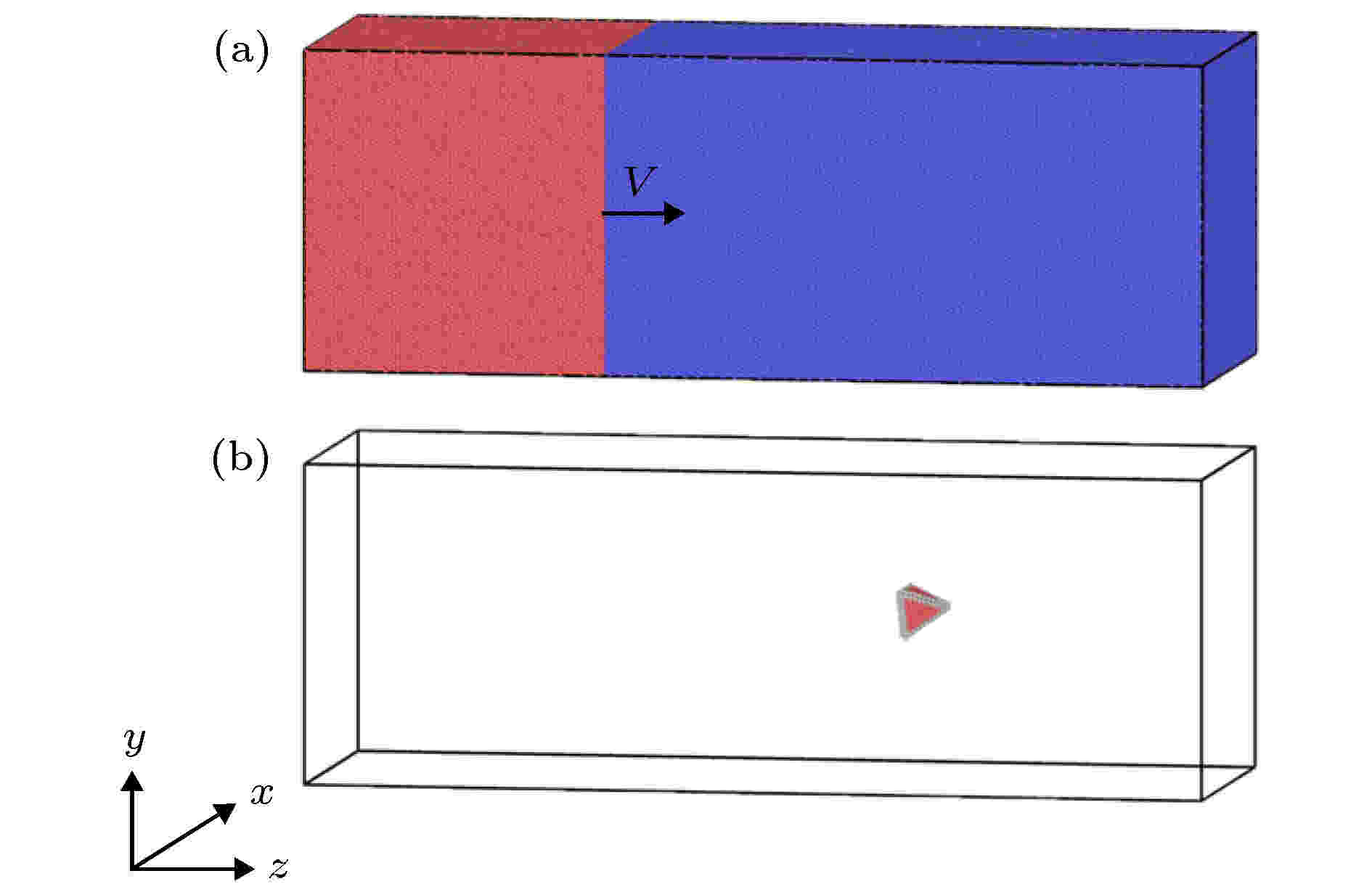2020, 69 (3): 036201. doi: 10.7498/aps.69.20191425

###### 凝聚物质：电子结构、电学、磁学和光学性质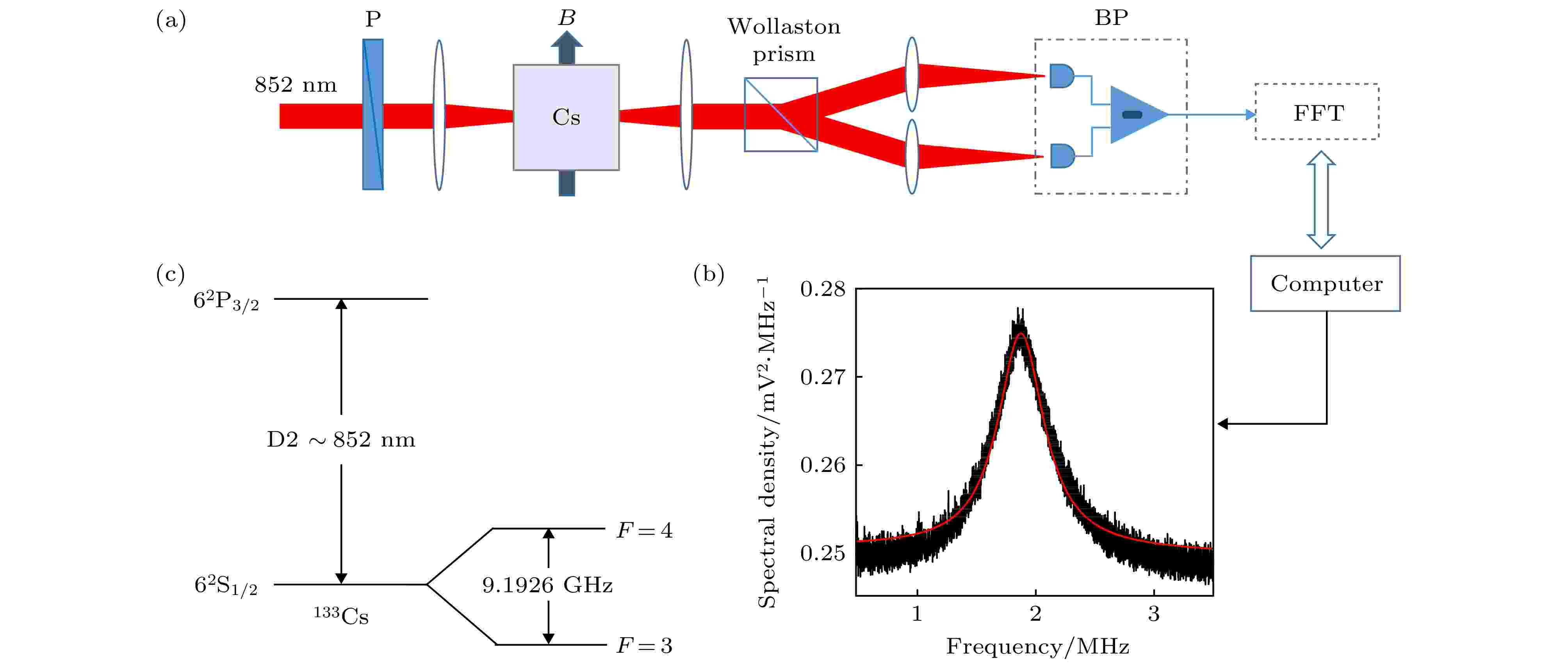## 编辑推荐

2020, 69 (3): 037201. doi: 10.7498/aps.69.20191623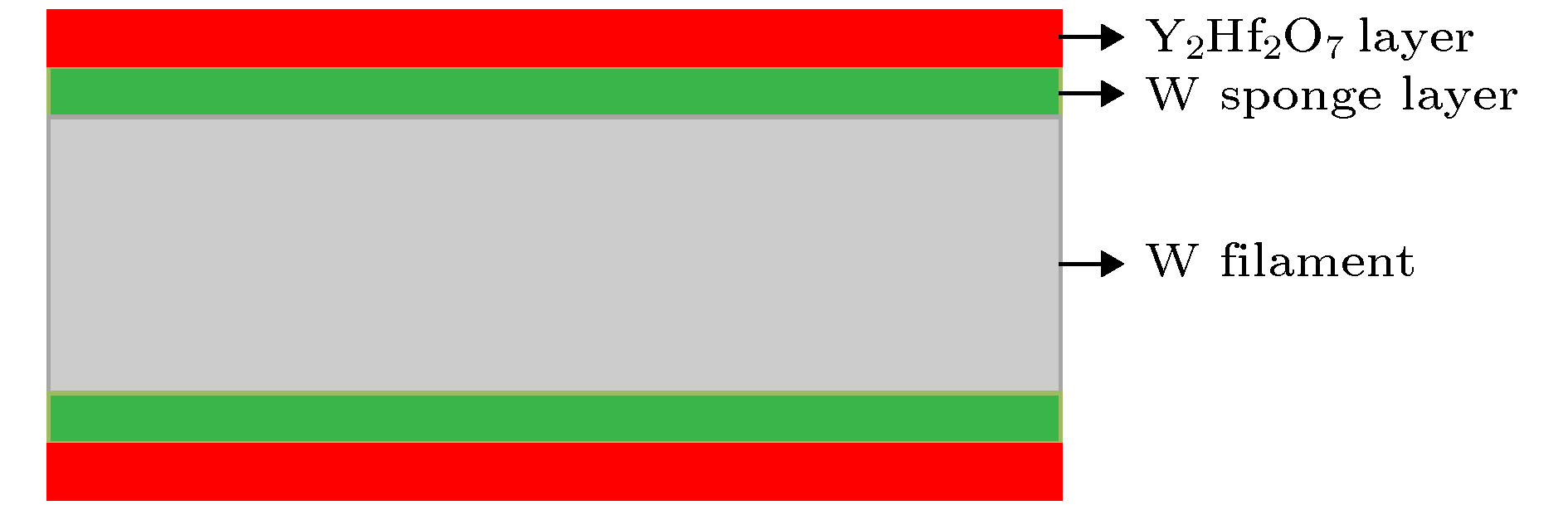2020, 69 (3): 037901. doi: 10.7498/aps.69.20191496

###### 物理学交叉学科及有关科学技术领域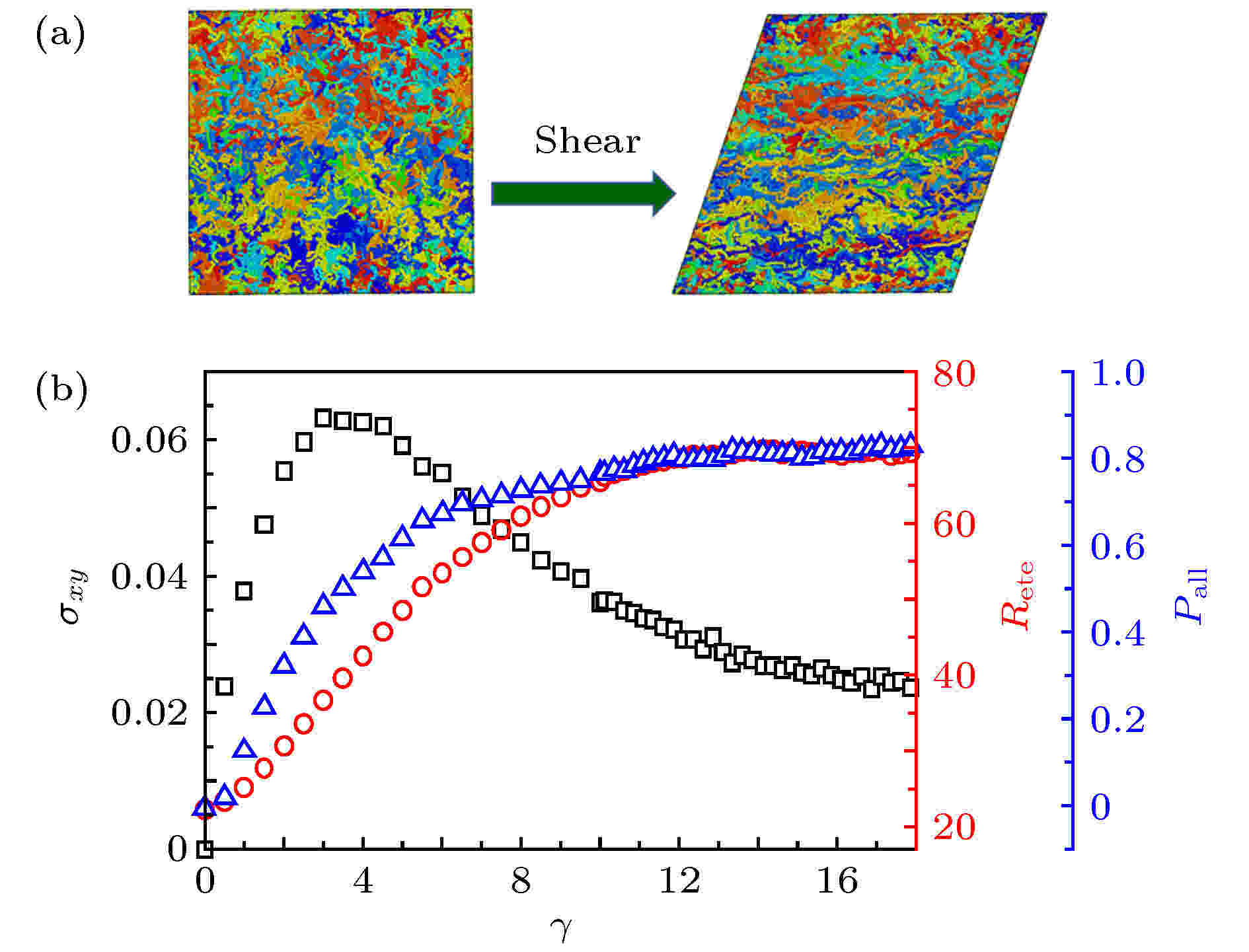2020, 69 (3): 038101. doi: 10.7498/aps.69.20191191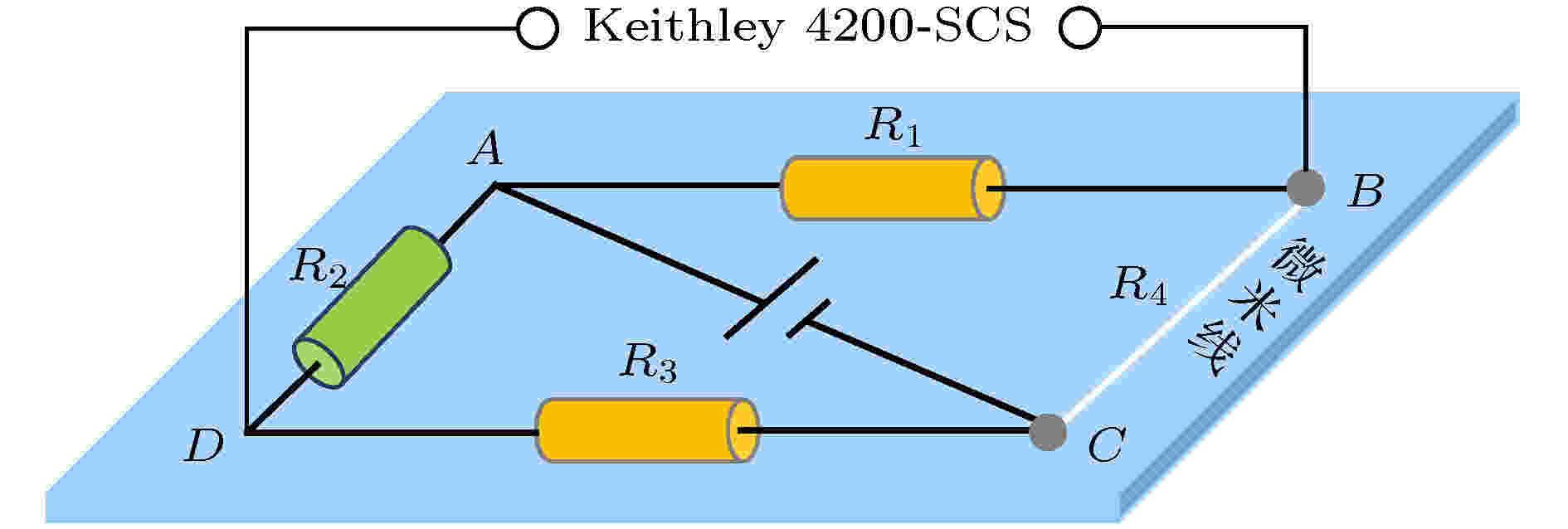2020, 69 (3): 038102. doi: 10.7498/aps.69.20191530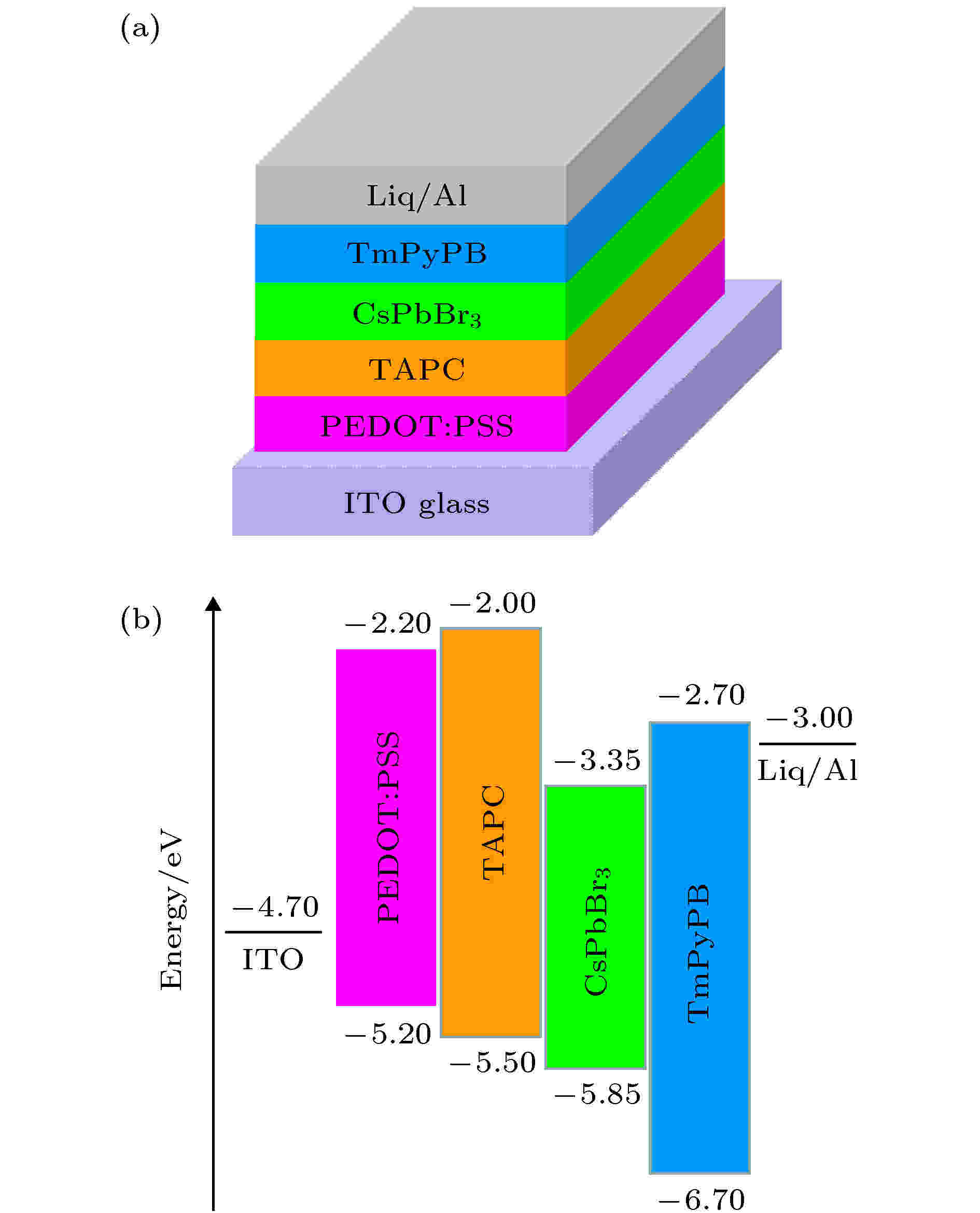## 编辑推荐

2020, 69 (3): 038501. doi: 10.7498/aps.69.20191263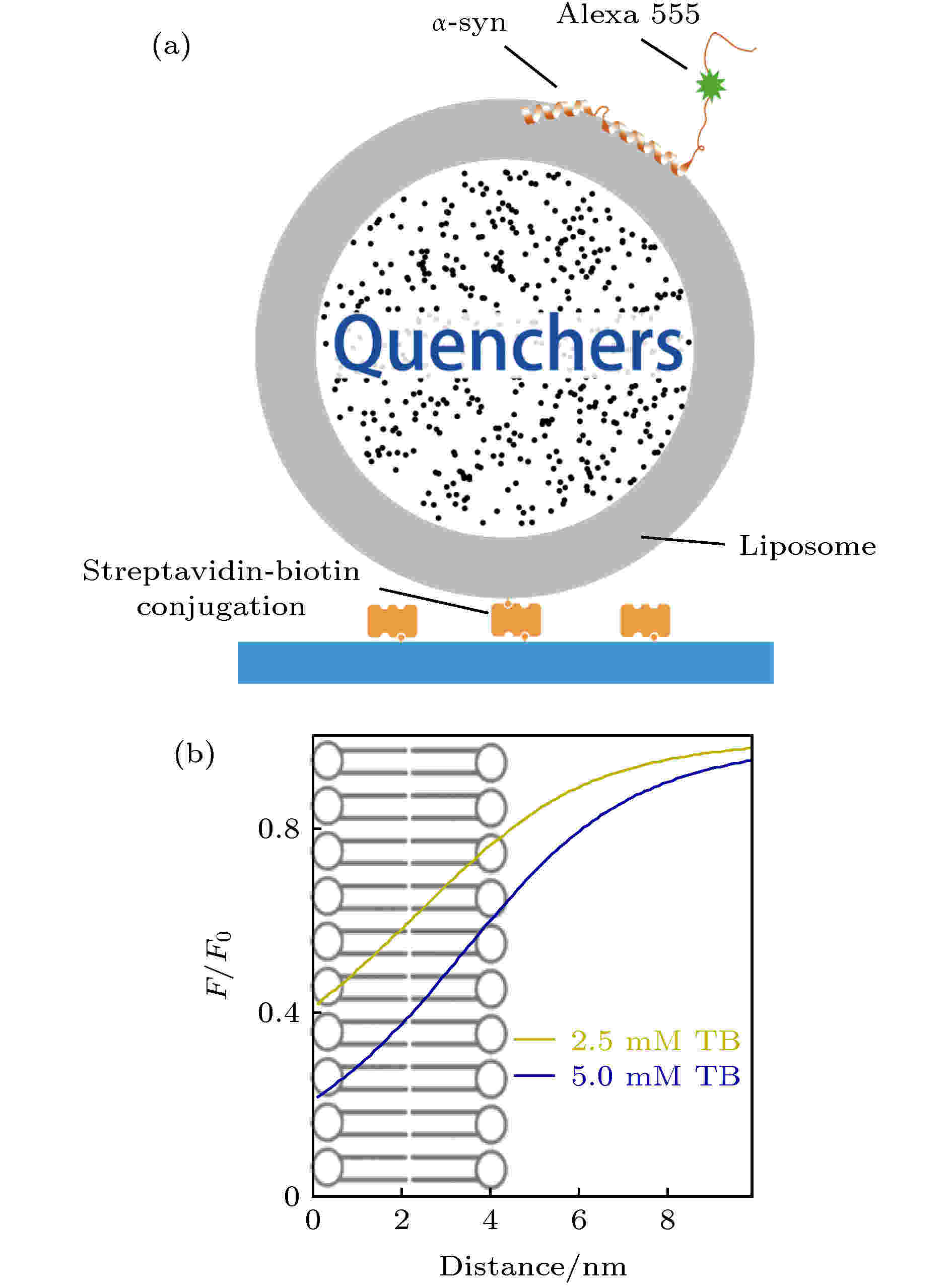## 编辑推荐

2020, 69 (3): 038701. doi: 10.7498/aps.69.20191607

α-突触核蛋白(α-synuclein, α-syn)在帕金森病的发病机理中起着关键的作用, 因而近年来受到了越来越广泛的关注. α-syn在膜上的动力学过程对理解其功能至关重要. 本文使用基于脂质体的单分子荧光衰减方法—LipoFRET, 首次对较高浓度下α-syn与磷脂膜的相互作用的动态过程进行了单分子层面上的研究. 研究发现, 在溶液中α-syn浓度升高时, 其中央NAC区域可离开磷脂膜表面进入水相中; 而N端部分位于膜表面内的位置变浅, 并有更高的概率脱离膜表面进入溶液中. 利用单分子荧光成像对α-syn解离的观察则发现, 随着溶液中的α-syn浓度升高, 脂质体上的α-syn解离速率加快. 因此高浓度下, α-syn在膜上各区域垂直位置变化促进了蛋白从膜上的解离. 结合LipoFRET的实验结果可以推断, α-syn的解离可能是由于不同的α-syn分子膜作用位点互相竞争而导致的解离. 这样的特征, 可能是体内环境中影响α-syn控制其聚集的重要性质.